# ML Aggarwal Solutions for Class 8 Maths Chapter 11 Factorisation

ML Aggarwal Solutions for Class 8 Maths Chapter 11 Factorisation are provided here to help students comprehend the concepts easily and secure high marks in exams. This chapter mainly deals with problems based on factorising polynomials using algebraic identities. For students who are aiming to secure top scores in their examinations, ML Aggarwal Solutions serves the purpose of the best reference material. These solutions are designed by individual subject matter experts who have vast academic experience, according to the latest ICSE guidelines. Further, these solutions are designed in a unique and simple method for every student to understand with ease. The PDF of solutions of this chapter is available here and can be downloaded from the link given below.

Chapter 11 Factorisation contains five exercises and Check your progress questions for which the ML Aggarwal Class 8 Solutions present in this page provides precise solutions. Using this resource on a daily basis can improve the problem-solving abilities of students, which are important from the examination point of view.

## Download the PDF of ML Aggarwal Solutions for Class 8 Maths Chapter 11 Factorisation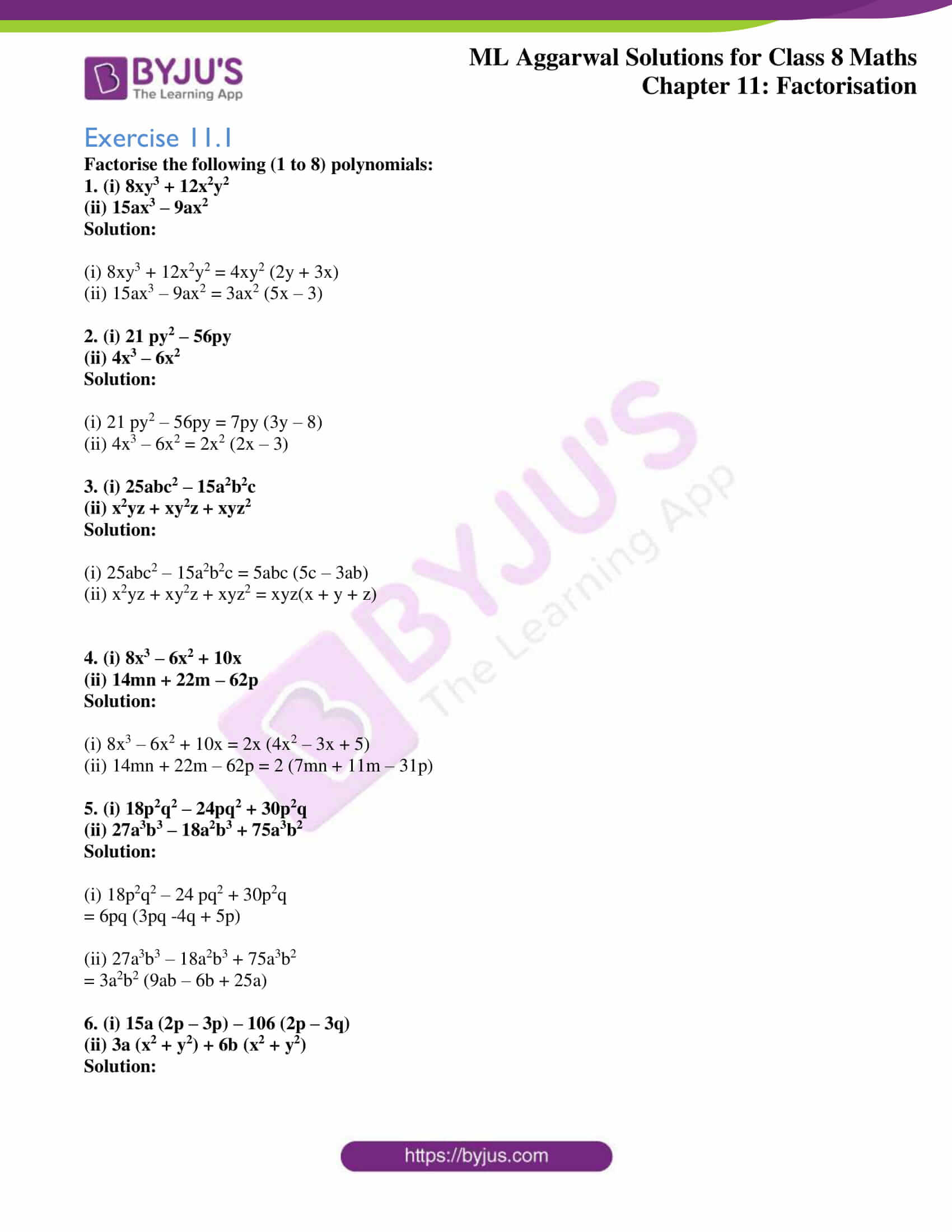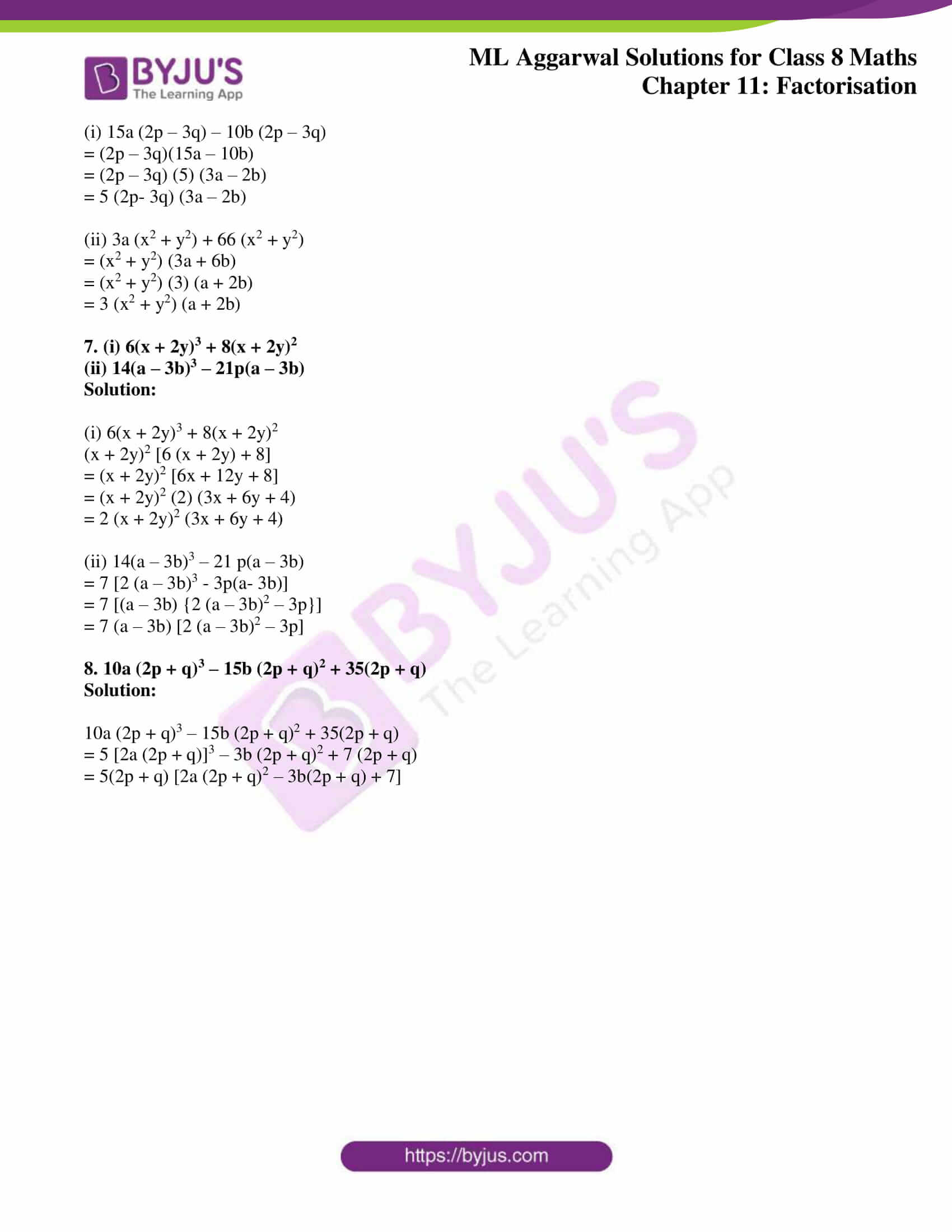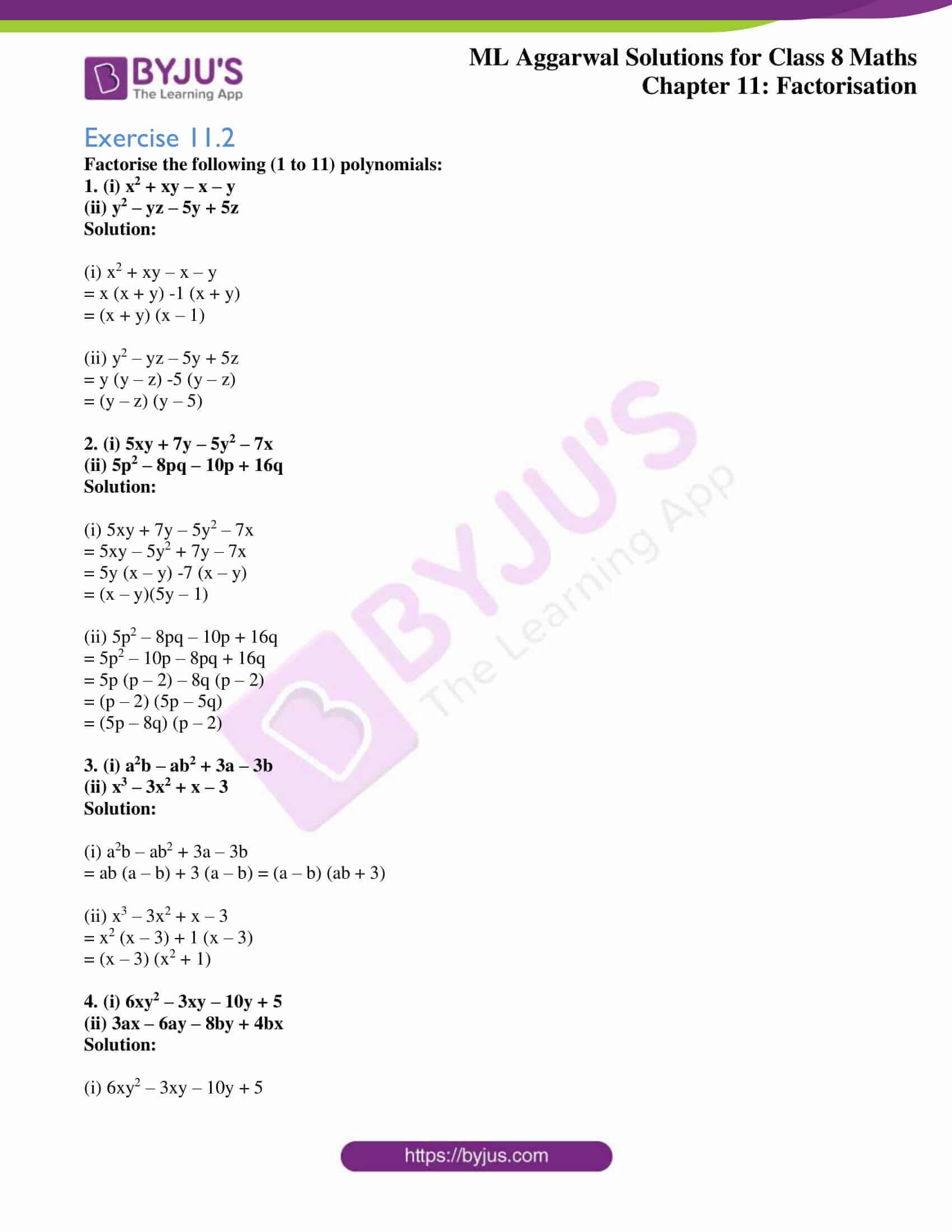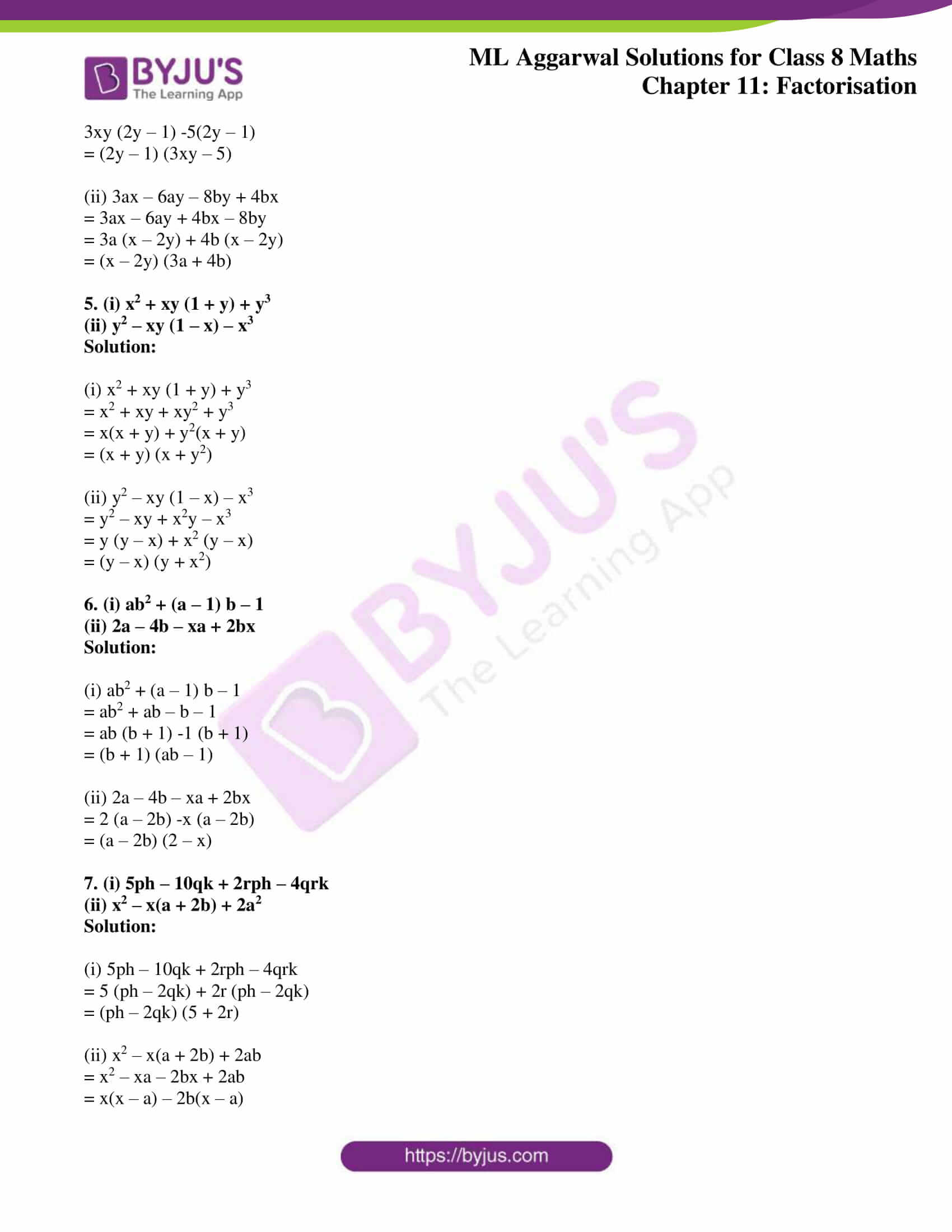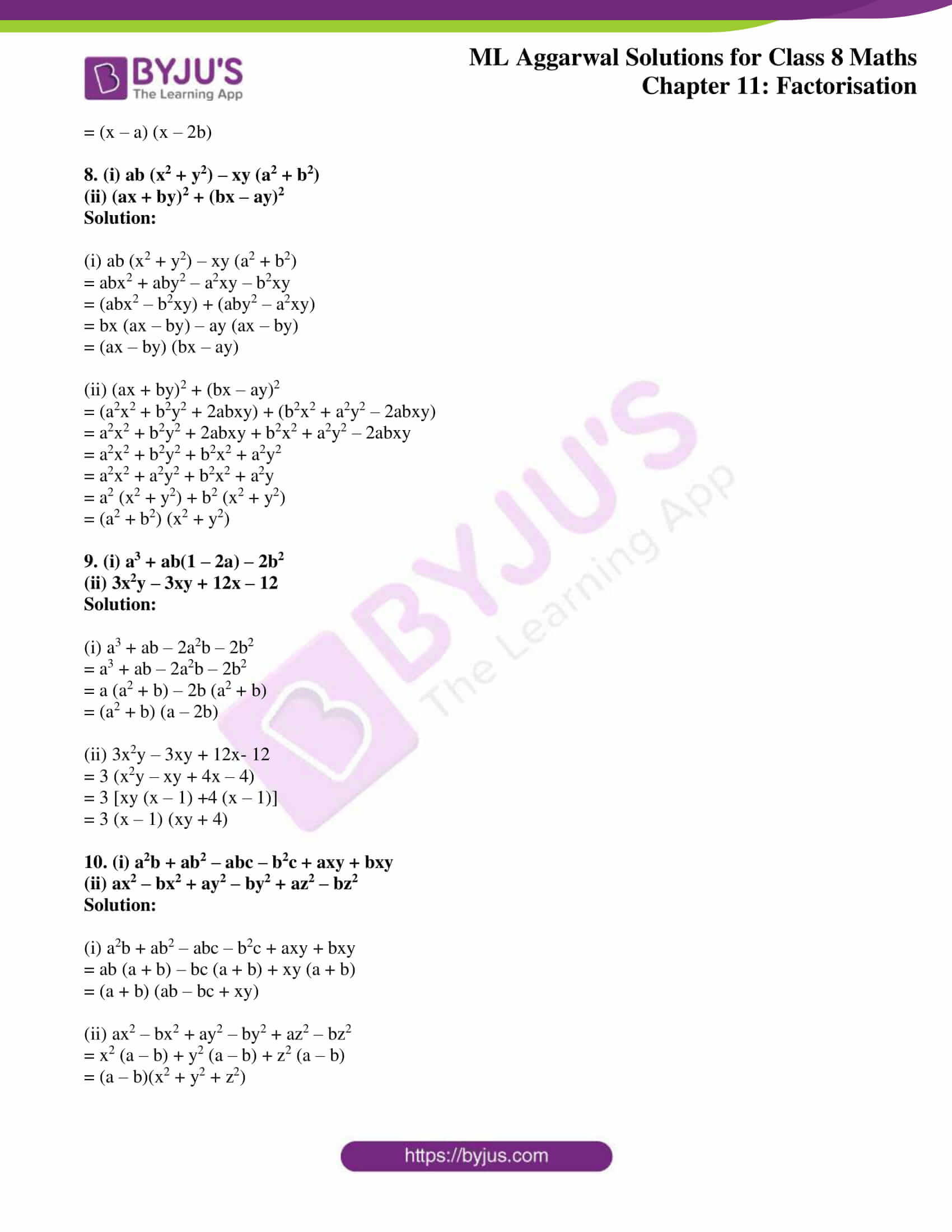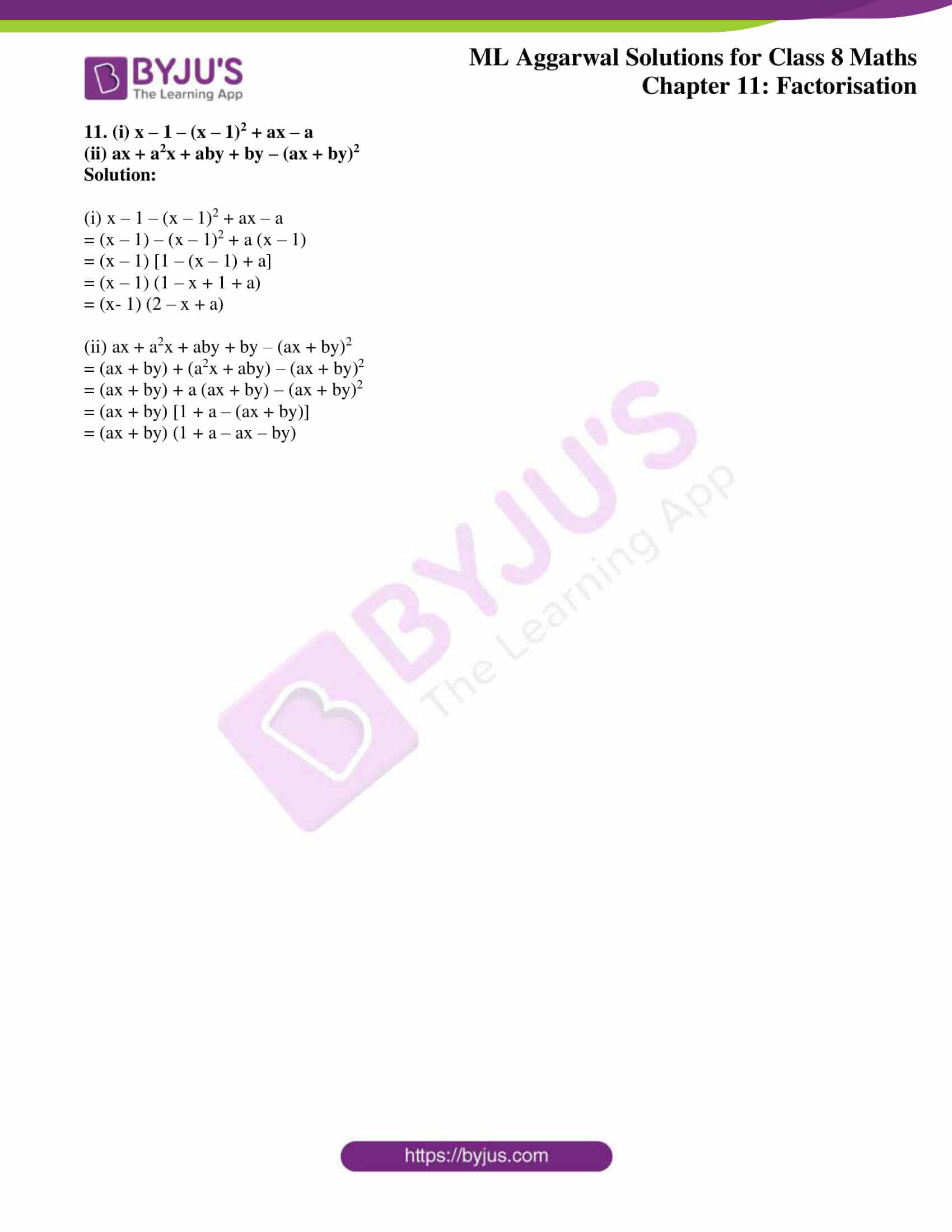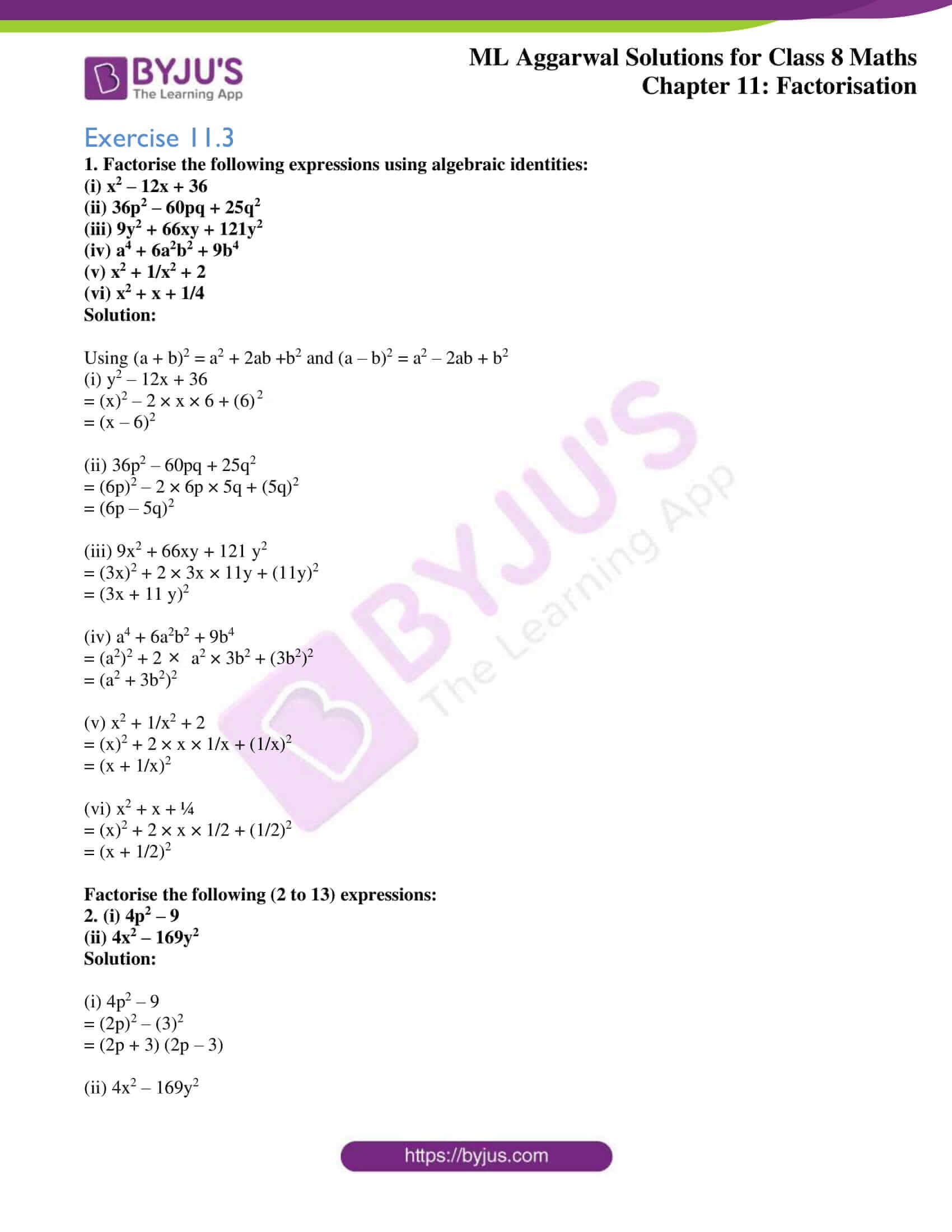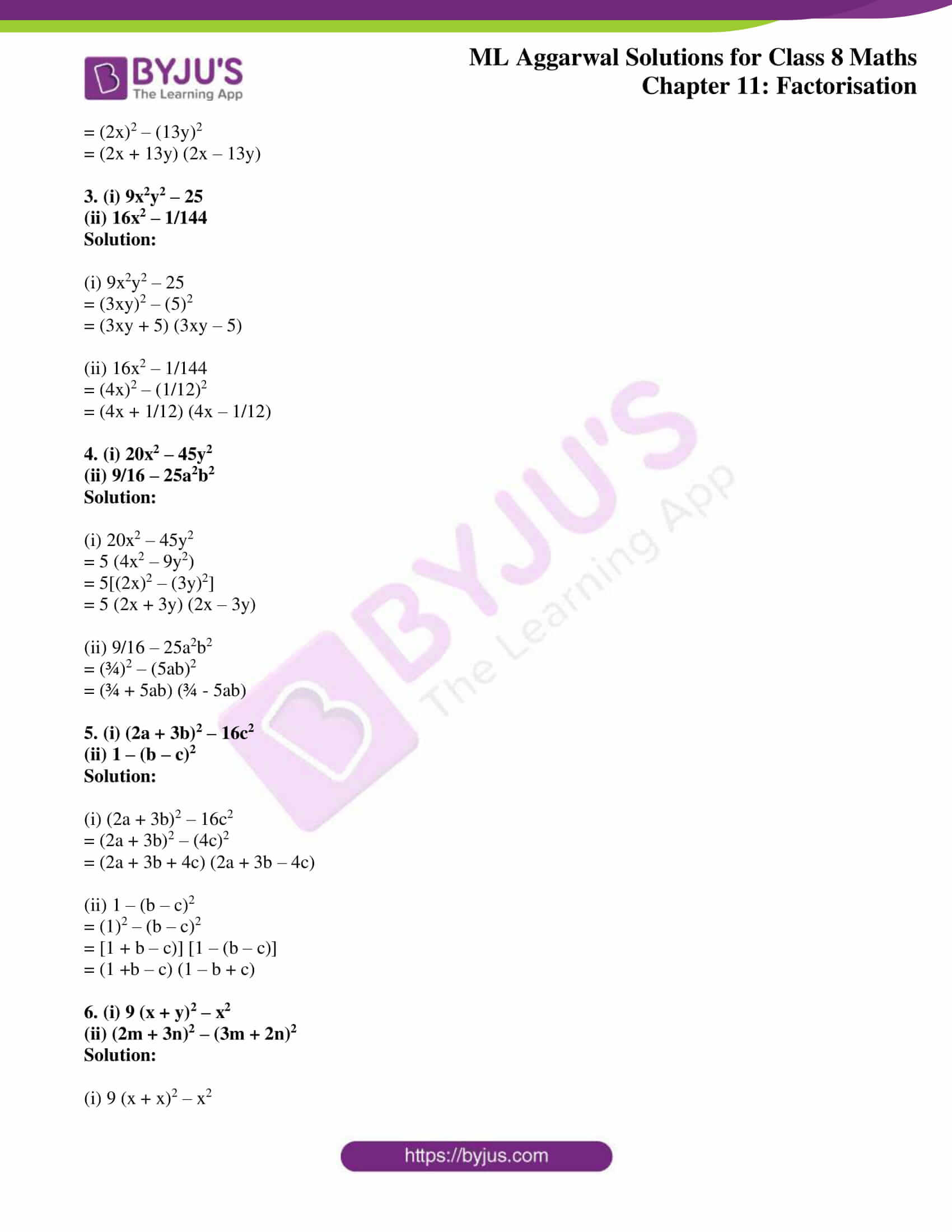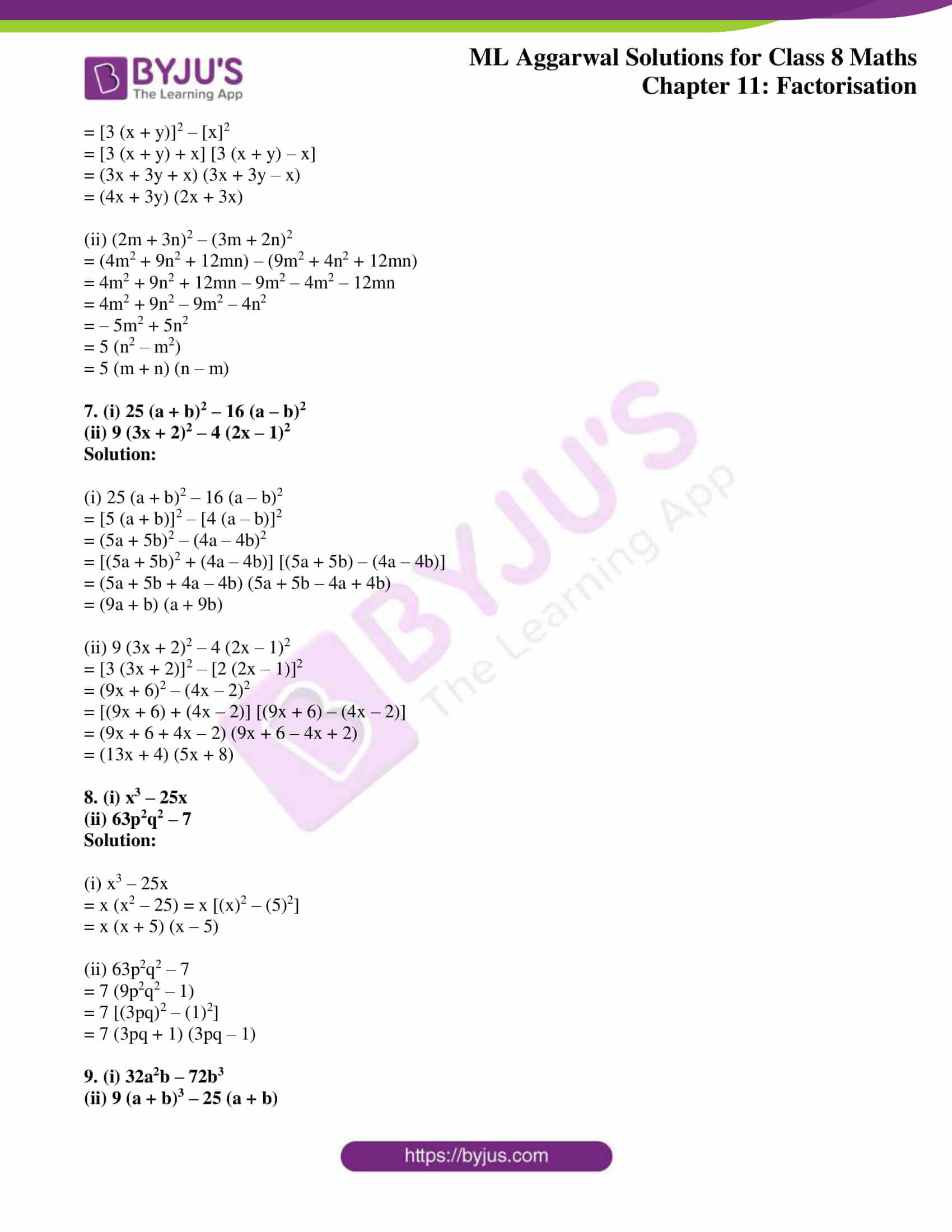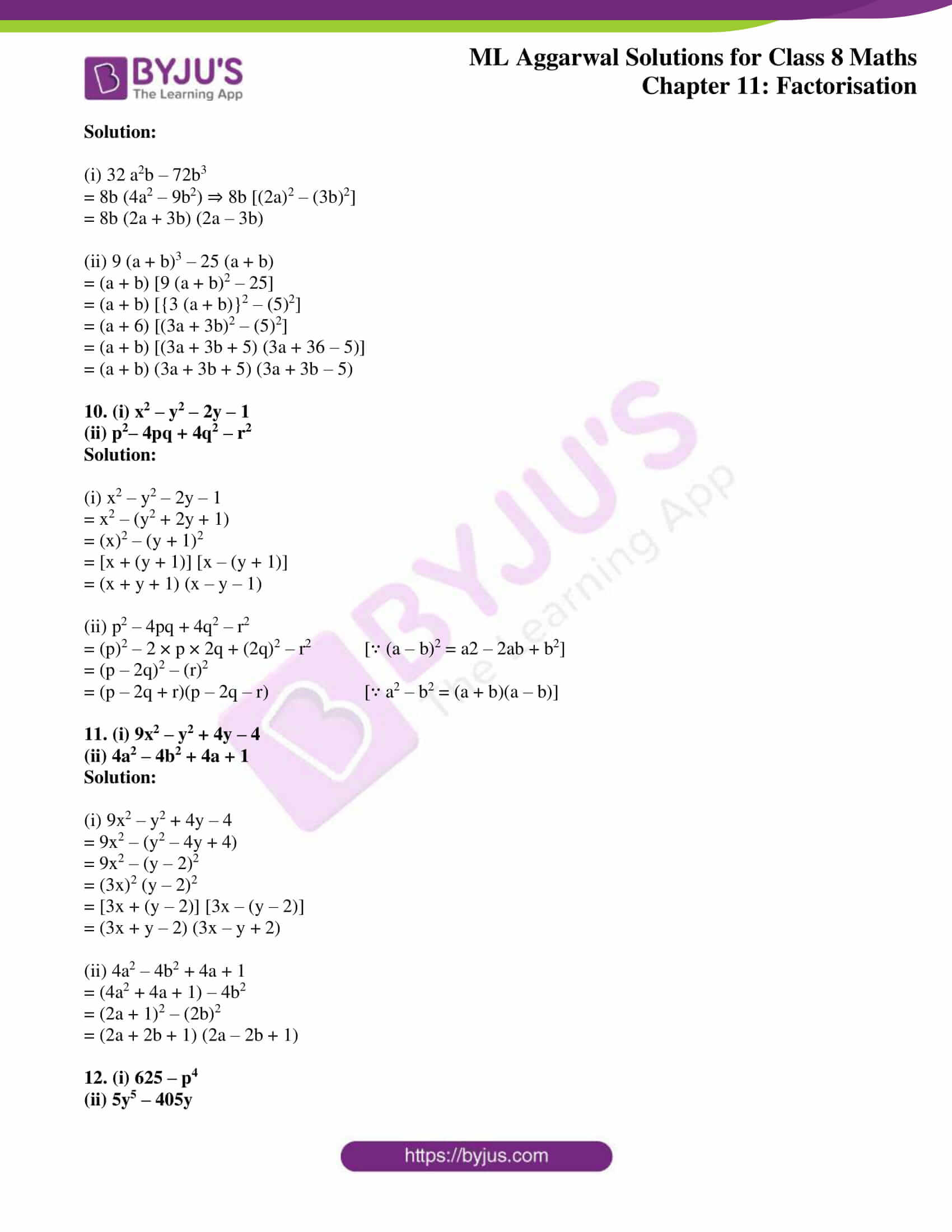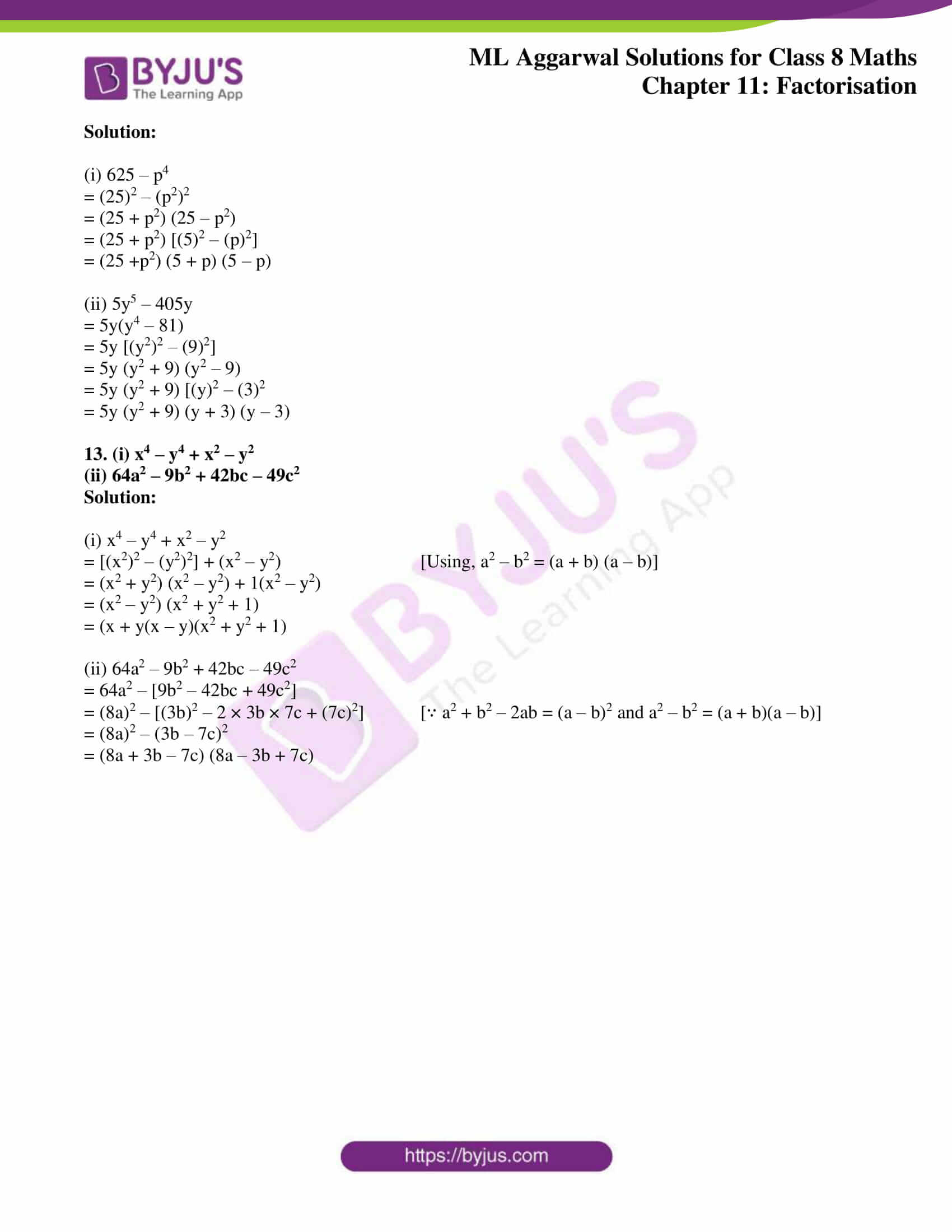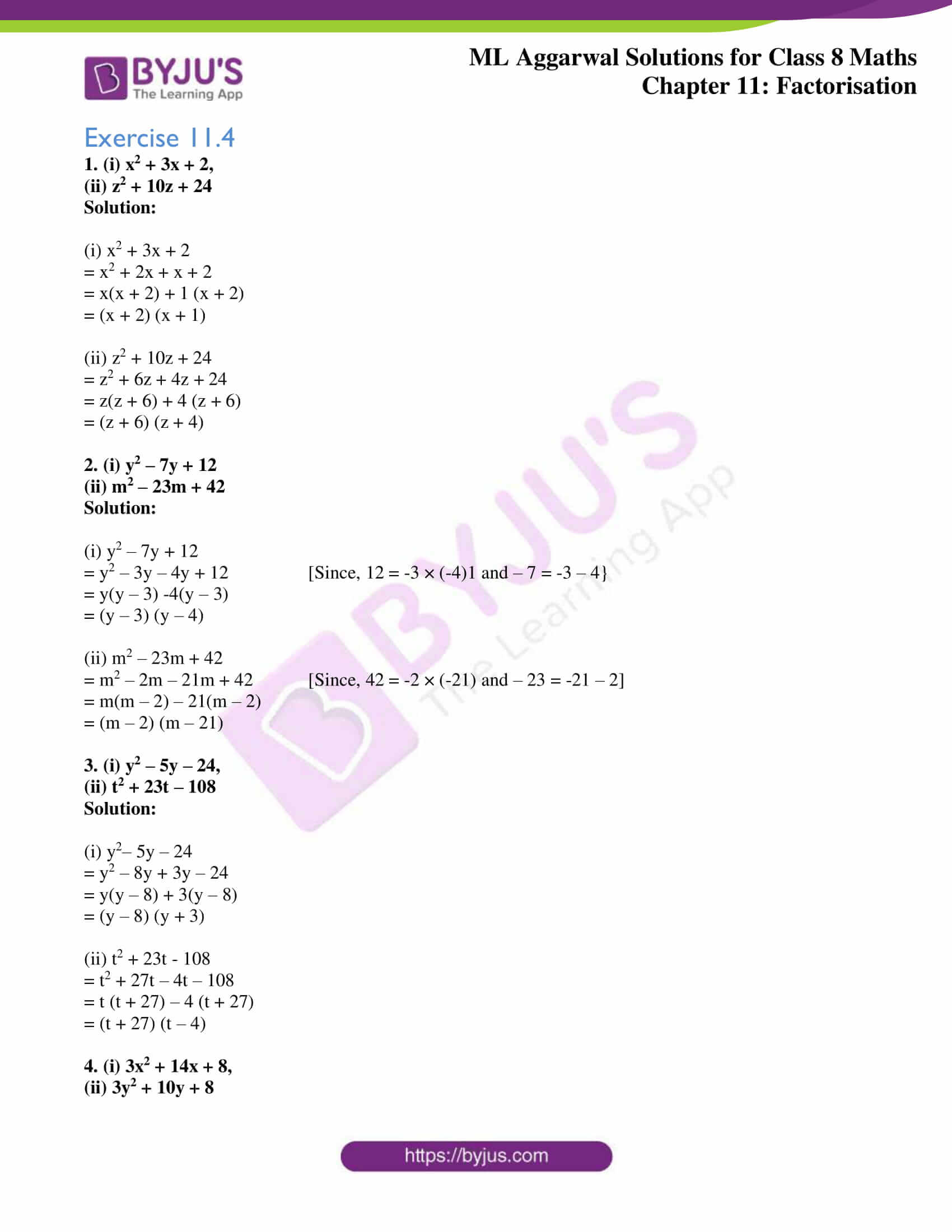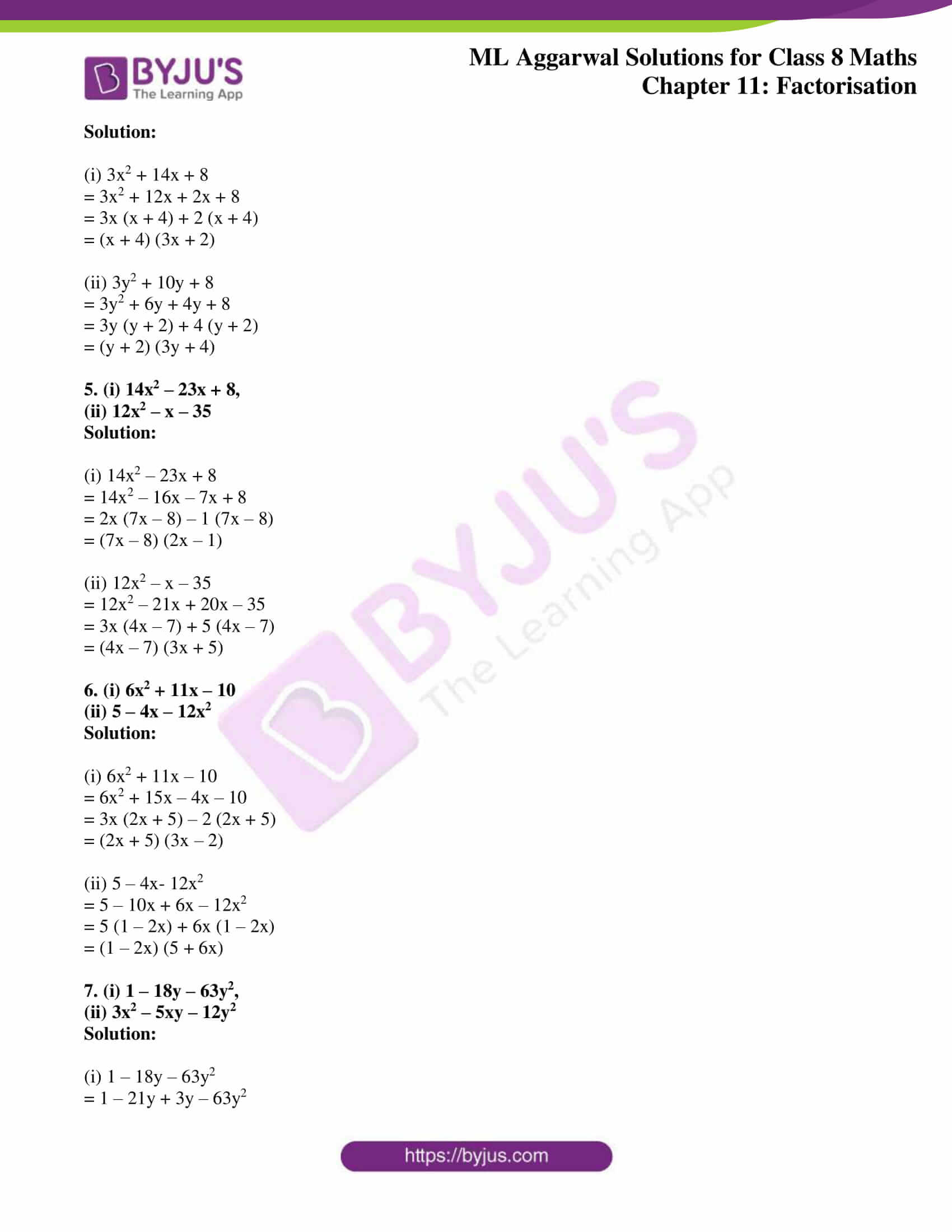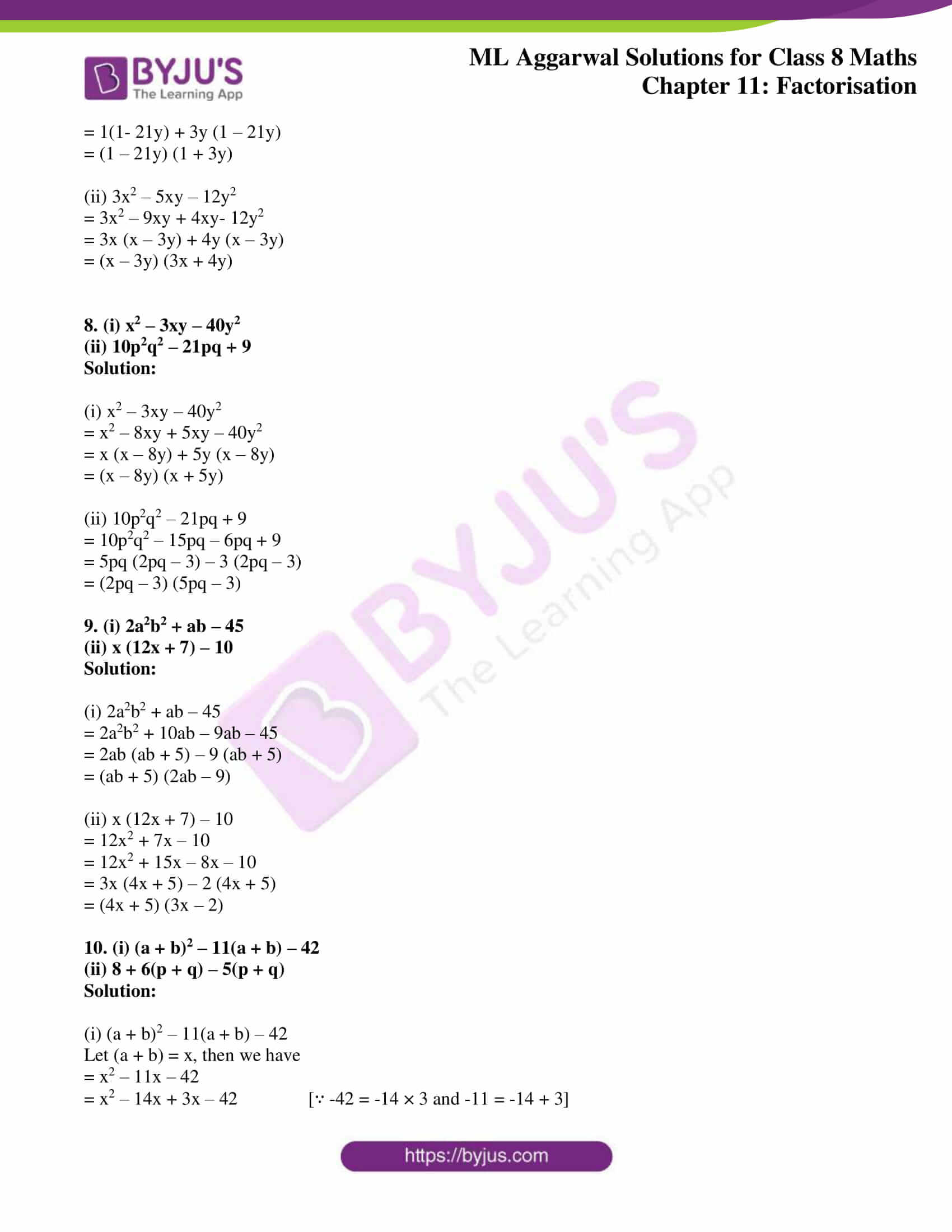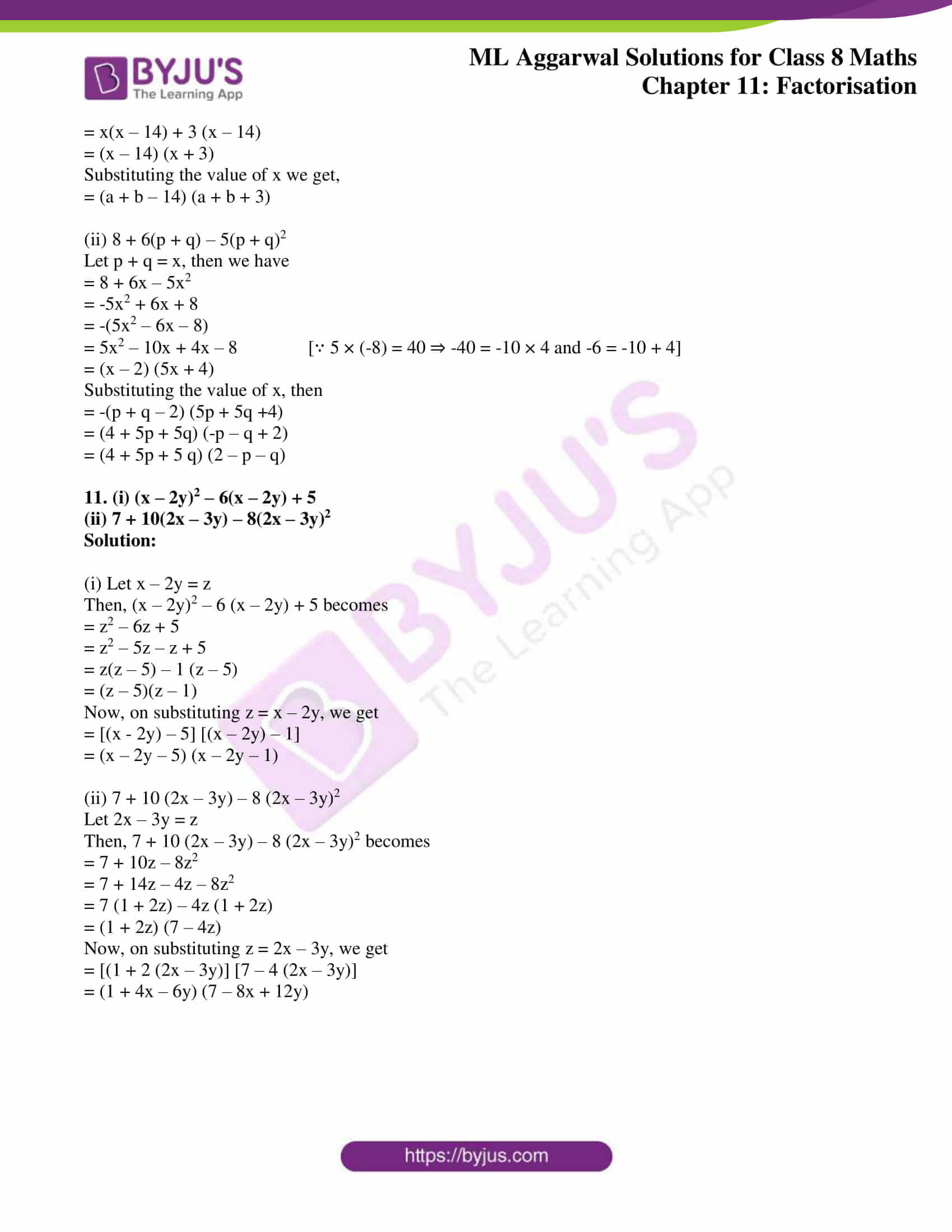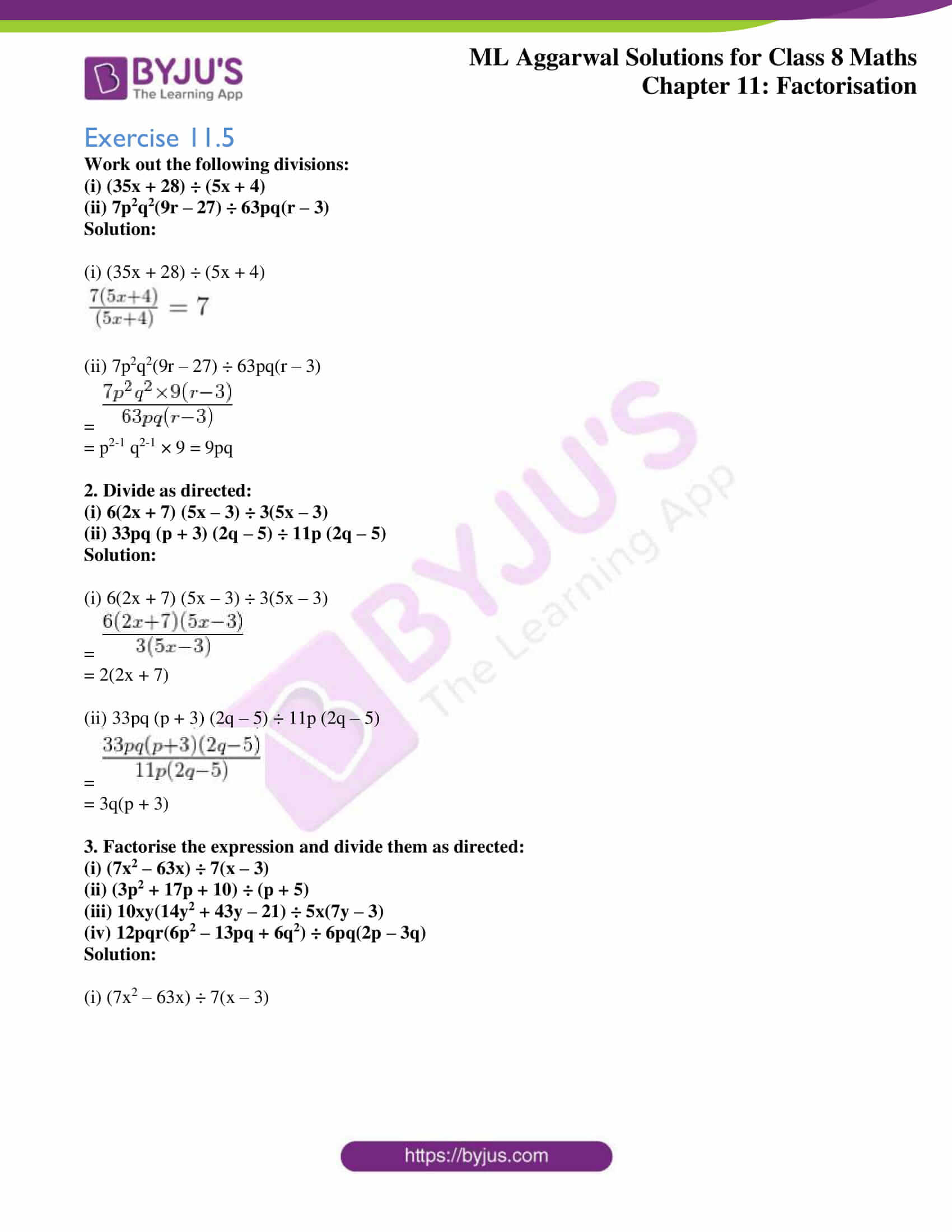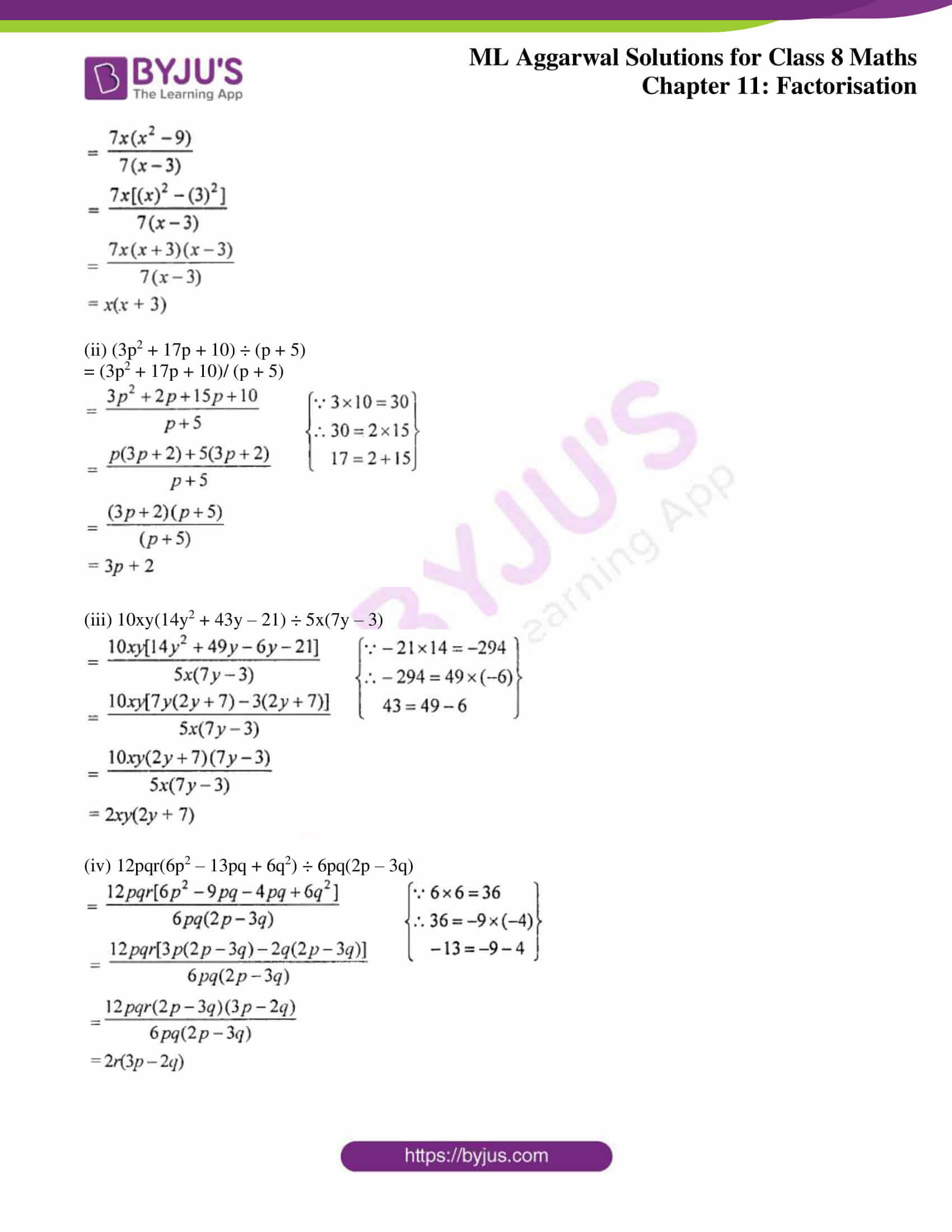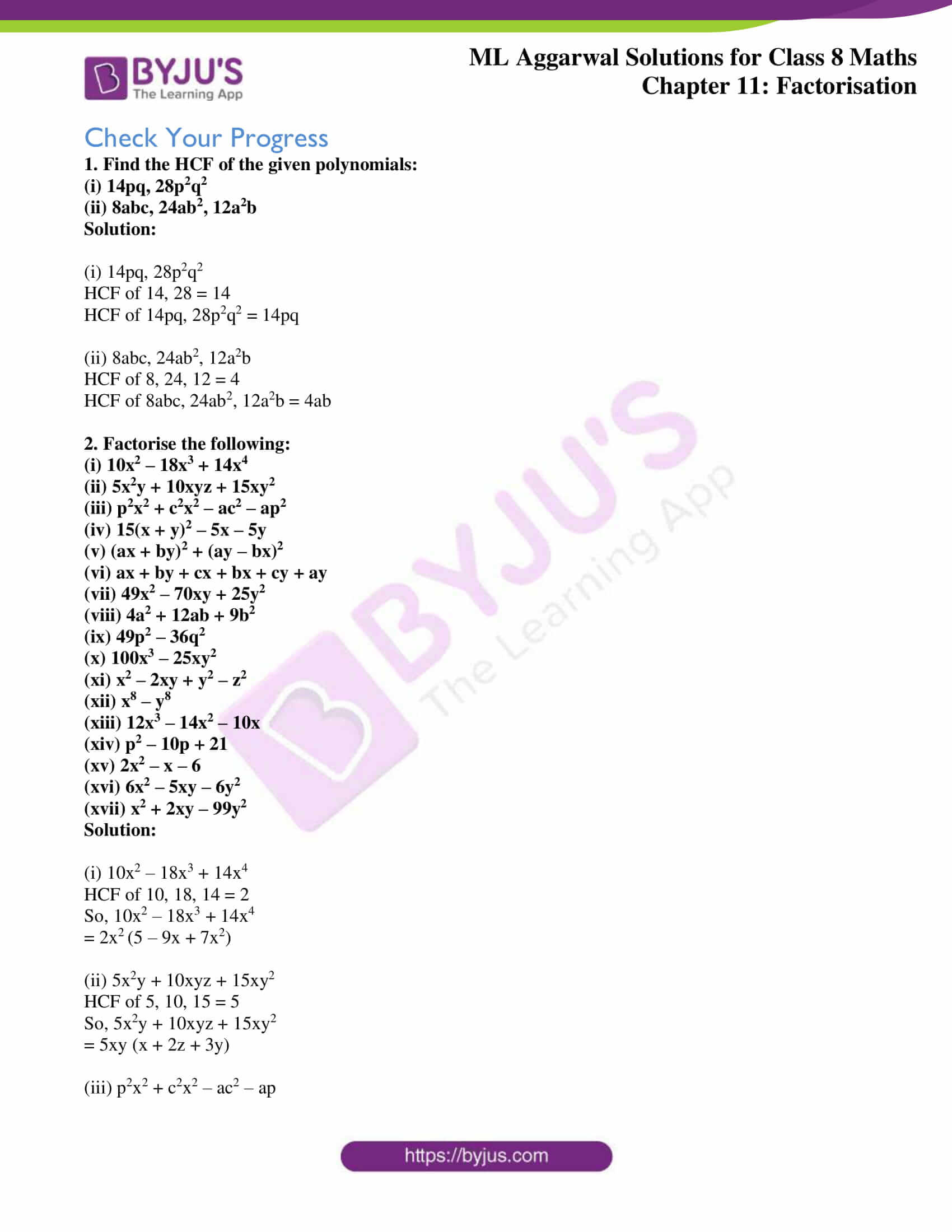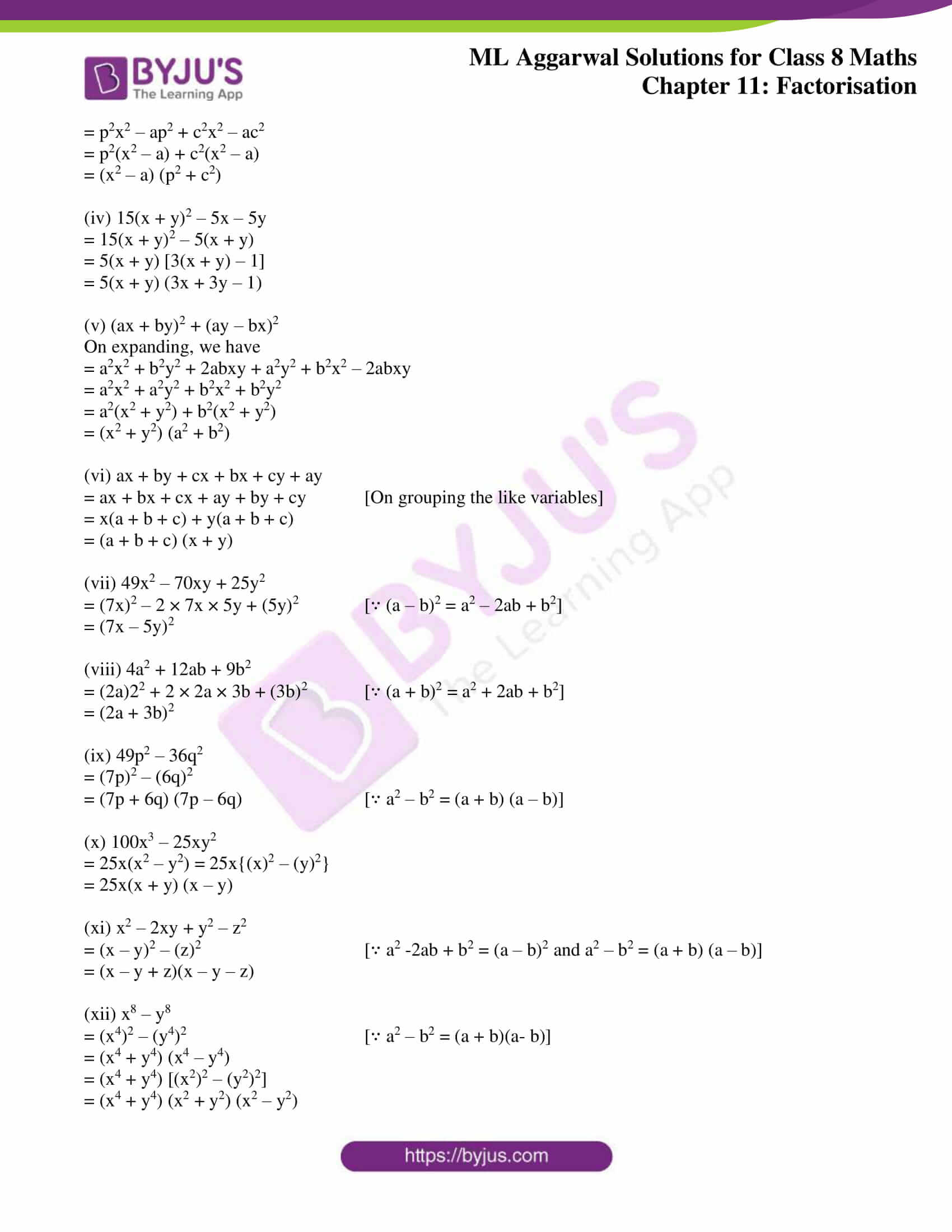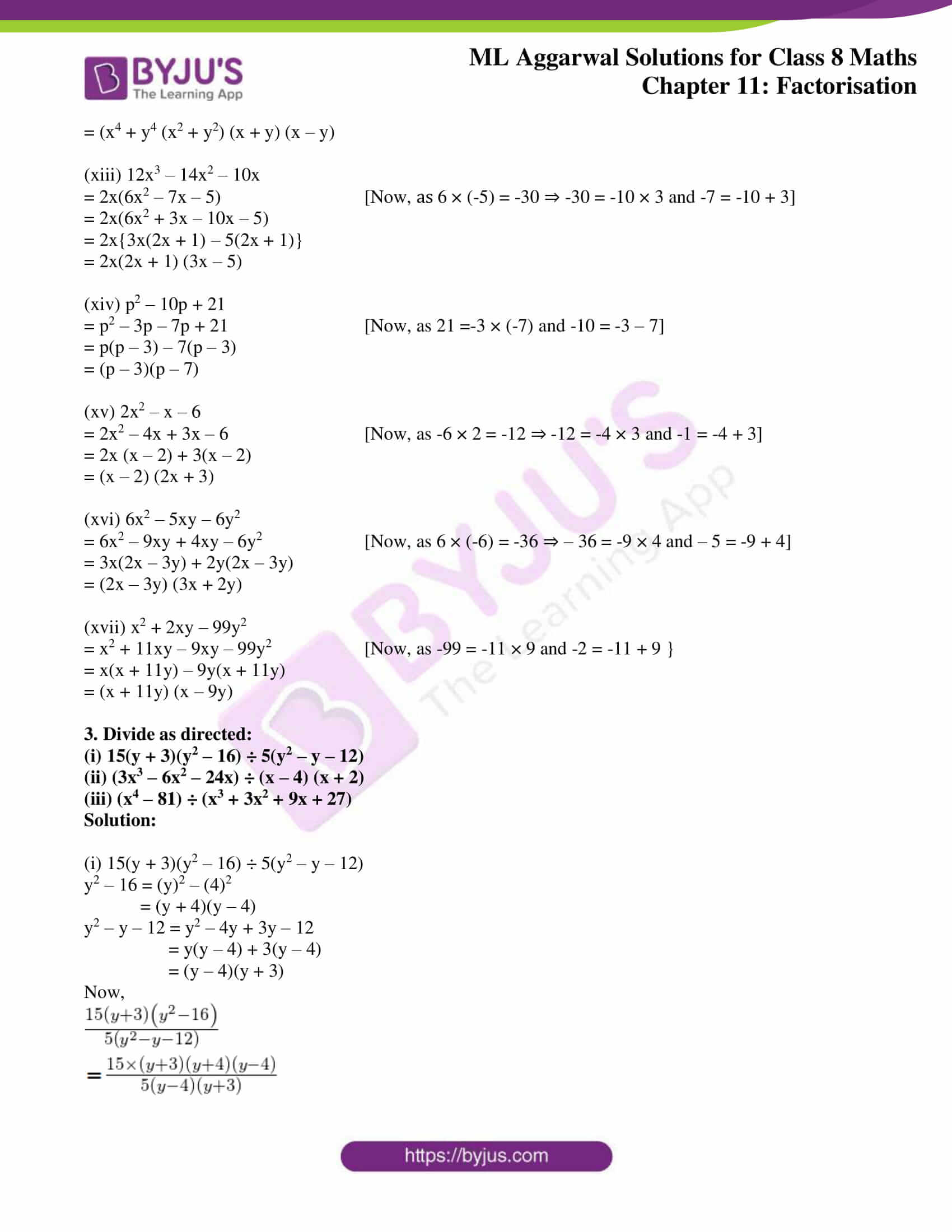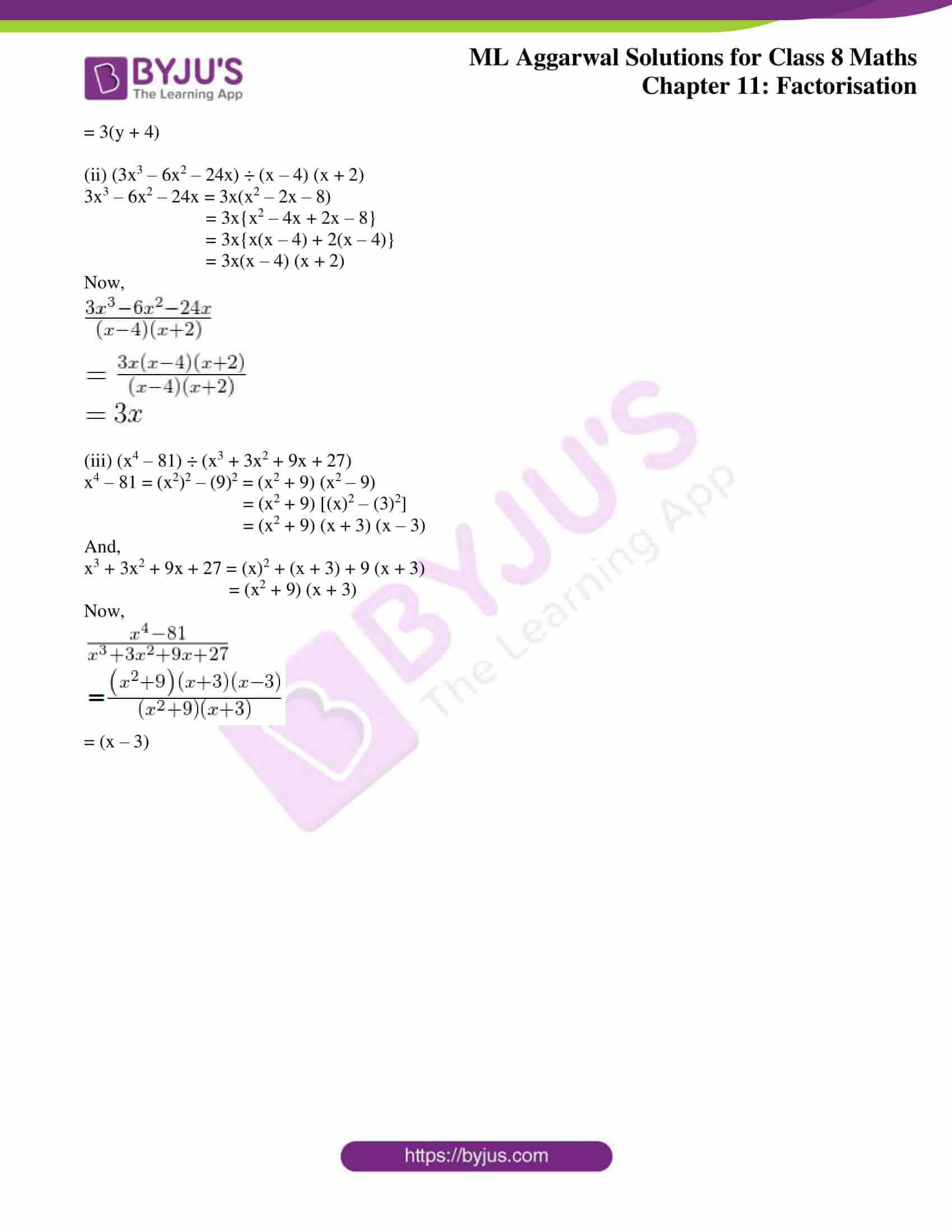### Access answers to ML Aggarwal Solutions for Class 8 Maths Chapter 11 Factorisation

Exercise 11.1

Factorise the following (1 to 8) polynomials:
1. (i) 8xy3 + 12x2y2
(ii) 15ax3 – 9ax2
Solution:

(i) 8xy3 + 12x2y2 = 4xy2 (2y + 3x)

(ii) 15ax3 – 9ax2 = 3ax2 (5x – 3)

2. (i) 21 py2 – 56py
(ii) 4x3 – 6x2
Solution:

(i) 21 py2 – 56py = 7py (3y – 8)

(ii) 4x3 – 6x2 = 2x2 (2x – 3)

3. (i) 25abc2 – 15a2b2c
(ii) x2yz + xy2z + xyz2
Solution:

(i) 25abc2 – 15a2b2c = 5abc (5c – 3ab)

(ii) x2yz + xy2z + xyz2 = xyz(x + y + z)

4. (i) 8x3 – 6x2 + 10x
(ii) 14mn + 22m – 62p
Solution:

(i) 8x3 – 6x2 + 10x = 2x (4x2 – 3x + 5)

(ii) 14mn + 22m – 62p = 2 (7mn + 11m – 31p)

5. (i) 18p2q2 – 24pq2 + 30p2q
(ii) 27a3b3 – 18a2b3 + 75a3b2
Solution:

(i) 18p2q2 – 24 pq2 + 30p2q

= 6pq (3pq -4q + 5p)

(ii) 27a3b3 – 18a2b3 + 75a3b2

= 3a2b2 (9ab – 6b + 25a)

6. (i) 15a (2p – 3p) – 106 (2p – 3q)
(ii) 3a (x2 + y2) + 6b (x2 + y2)
Solution:

(i) 15a (2p – 3q) – 10b (2p – 3q)

= (2p – 3q)(15a – 10b)

= (2p – 3q) (5) (3a – 2b)

= 5 (2p- 3q) (3a – 2b)

(ii) 3a (x2 + y2) + 66 (x2 + y2)

= (x2 + y2) (3a + 6b)

= (x2 + y2) (3) (a + 2b)

= 3 (x2 + y2) (a + 2b)

7. (i) 6(x + 2y)3 + 8(x + 2y)2
(ii) 14(a – 3b)3 – 21p(a – 3b)
Solution:

(i) 6(x + 2y)3 + 8(x + 2y)2

(x + 2y)2 [6 (x + 2y) + 8]

= (x + 2y)2 [6x + 12y + 8]

= (x + 2y)2 (2) (3x + 6y + 4)

= 2 (x + 2y)2 (3x + 6y + 4)

(ii) 14(a – 3b)3 – 21 p(a – 3b)

= 7 [2 (a – 3b)3 – 3p(a- 3b)]

= 7 [(a – 3b) {2 (a – 3b)2 – 3p}]

= 7 (a – 3b) [2 (a – 3b)2 – 3p]

8. 10a (2p + q)3 – 15b (2p + q)2 + 35(2p + q)
Solution:

10a (2p + q)3 – 15b (2p + q)2 + 35(2p + q)

= 5 [2a (2p + q)]3 – 3b (2p + q)2 + 7 (2p + q)

= 5(2p + q) [2a (2p + q)2 – 3b(2p + q) + 7]

Exercise 11.2

Factorise the following (1 to 11) polynomials:
1. (i) x2 + xy – x – y
(ii) y2 – yz – 5y + 5z
Solution:

(i) x2 + xy – x – y

= x (x + y) -1 (x + y)

= (x + y) (x – 1)

(ii) y2 – yz – 5y + 5z

= y (y – z) -5 (y – z)

= (y – z) (y – 5)

2. (i) 5xy + 7y – 5y2 – 7x
(ii) 5p2 – 8pq – 10p + 16q
Solution:

(i) 5xy + 7y – 5y2 – 7x

= 5xy – 5y2 + 7y – 7x

= 5y (x – y) -7 (x – y)

= (x – y)(5y – 1)

(ii) 5p2 – 8pq – 10p + 16q

= 5p2 – 10p – 8pq + 16q

= 5p (p – 2) – 8q (p – 2)

= (p – 2) (5p – 5q)

= (5p – 8q) (p – 2)

3. (i) a2b – ab2 + 3a – 3b
(ii) x3 – 3x2 + x – 3
Solution:

(i) a2b – ab2 + 3a – 3b

= ab (a – b) + 3 (a – b) = (a – b) (ab + 3)

(ii) x3 – 3x2 + x – 3

= x2 (x – 3) + 1 (x – 3)

= (x – 3) (x2 + 1)

4. (i) 6xy2 – 3xy – 10y + 5
(ii) 3ax – 6ay – 8by + 4bx
Solution:

(i) 6xy2 – 3xy – 10y + 5

3xy (2y – 1) -5(2y – 1)

= (2y – 1) (3xy – 5)

(ii) 3ax – 6ay – 8by + 4bx

= 3ax – 6ay + 4bx – 8by

= 3a (x – 2y) + 4b (x – 2y)

= (x – 2y) (3a + 4b)

5. (i) x2 + xy (1 + y) + y3
(ii) y2 – xy (1 – x) – x3
Solution:

(i) x2 + xy (1 + y) + y3

= x2 + xy + xy2 + y3

= x(x + y) + y2(x + y)

= (x + y) (x + y2)

(ii) y2 – xy (1 – x) – x3

= y2 – xy + x2y – x3

= y (y – x) + x2 (y – x)

= (y – x) (y + x2)

6. (i) ab2 + (a – 1) b – 1
(ii) 2a – 4b – xa + 2bx
Solution:

(i) ab2 + (a – 1) b – 1

= ab2 + ab – b – 1

= ab (b + 1) -1 (b + 1)

= (b + 1) (ab – 1)

(ii) 2a – 4b – xa + 2bx

= 2 (a – 2b) -x (a – 2b)

= (a – 2b) (2 – x)

7. (i) 5ph – 10qk + 2rph – 4qrk
(ii) x2 – x(a + 2b) + 2a2
Solution:

(i) 5ph – 10qk + 2rph – 4qrk

= 5 (ph – 2qk) + 2r (ph – 2qk)

= (ph – 2qk) (5 + 2r)

(ii) x2 – x(a + 2b) + 2ab

= x2 – xa – 2bx + 2ab

= x(x – a) – 2b(x – a)

= (x – a) (x – 2b)

8. (i) ab (x2 + y2) – xy (a2 + b2)
(ii) (ax + by)2 + (bx – ay)2
Solution:

(i) ab (x2 + y2) – xy (a2 + b2)

= abx2 + aby2 – a2xy – b2xy

= (abx2 – b2xy) + (aby2 – a2xy)

= bx (ax – by) – ay (ax – by)

= (ax – by) (bx – ay)

(ii) (ax + by)2 + (bx – ay)2

= (a2x2 + b2y2 + 2abxy) + (b2x2 + a2y2 – 2abxy)

= a2x2 + b2y2 + 2abxy + b2x2 + a2y2 – 2abxy

= a2x2 + b2y2 + b2x2 + a2y2

= a2x2 + a2y2 + b2x2 + a2y

= a2 (x2 + y2) + b2 (x2 + y2)

= (a2 + b2) (x2 + y2)

9. (i) a3 + ab(1 – 2a) – 2b2
(ii) 3x2y – 3xy + 12x – 12
Solution:

(i) a3 + ab – 2a2b – 2b2

= a3 + ab – 2a2b – 2b2

= a (a2 + b) – 2b (a2 + b)

= (a2 + b) (a – 2b)

(ii) 3x2y – 3xy + 12x- 12

= 3 (x2y – xy + 4x – 4)

= 3 [xy (x – 1) +4 (x – 1)]

= 3 (x – 1) (xy + 4)

10. (i) a2b + ab2 – abc – b2c + axy + bxy
(ii) ax2 – bx2 + ay2 – by2 + az2 – bz2
Solution:

(i) a2b + ab2 – abc – b2c + axy + bxy

= ab (a + b) – bc (a + b) + xy (a + b)

= (a + b) (ab – bc + xy)

(ii) ax2 – bx2 + ay2 – by2 + az2 – bz2

= x2 (a – b) + y2 (a – b) + z2 (a – b)

= (a – b)(x2 + y2 + z2)

11. (i) x – 1 – (x – 1)2 + ax – a
(ii) ax + a2x + aby + by – (ax + by)2
Solution:

(i) x – 1 – (x – 1)2 + ax – a

= (x – 1) – (x – 1)2 + a (x – 1)

= (x – 1) [1 – (x – 1) + a]

= (x – 1) (1 – x + 1 + a)

= (x- 1) (2 – x + a)

(ii) ax + a2x + aby + by – (ax + by)2

= (ax + by) + (a2x + aby) – (ax + by)2

= (ax + by) + a (ax + by) – (ax + by)2

= (ax + by) [1 + a – (ax + by)]

= (ax + by) (1 + a – ax – by)

Exercise 11.3

1. Factorise the following expressions using algebraic identities:
(i) x2 – 12x + 36
(ii) 36p2 – 60pq + 25q2
(iii) 9y2 + 66xy + 121y2
(iv) a4 + 6a2b2 + 9b4
(v) x2 + 1/x2 + 2
(vi) x2 + x + 1/4
Solution:

Using (a + b)2 = a2 + 2ab +b2 and (a – b)2 = a2 – 2ab + b2

(i) y2 – 12x + 36

= (x)2 – 2 × x × 6 + (6)2

= (x – 6)2

(ii) 36p2 – 60pq + 25q2

= (6p)2 – 2 × 6p × 5q + (5q)2

= (6p – 5q)2

(iii) 9x2 + 66xy + 121 y2

= (3x)2 + 2 × 3x × 11y + (11y)2

= (3x + 11 y)2

(iv) a4 + 6a2b2 + 9b4

= (a2)2 + 2 × a2 × 3b2 + (3b2)2

= (a2 + 3b2)2

(v) x2 + 1/x2 + 2

= (x)2 + 2 × x × 1/x + (1/x)2

= (x + 1/x)2

(vi) x2 + x + ¼

= (x)2 + 2 × x × 1/2 + (1/2)2

= (x + 1/2)2

Factorise the following (2 to 13) expressions:
2. (i) 4p2 – 9
(ii) 4x2 – 169y2
Solution:

(i) 4p2 – 9

= (2p)2 – (3)2

= (2p + 3) (2p – 3)

(ii) 4x2 – 169y2

= (2x)2 – (13y)2

= (2x + 13y) (2x – 13y)

3. (i) 9x2y2 – 25
(ii) 16x2 – 1/144
Solution:

(i) 9x2y2 – 25

= (3xy)2 – (5)2

= (3xy + 5) (3xy – 5)

(ii) 16x2 – 1/144

= (4x)2 – (1/12)2

= (4x + 1/12) (4x – 1/12)

4. (i) 20x2 – 45y2
(ii) 9/16 – 25a2b2
Solution:

(i) 20x2 – 45y2

= 5 (4x2 – 9y2)

= 5[(2x)2 – (3y)2]

= 5 (2x + 3y) (2x – 3y)

(ii) 9/16 – 25a2b2

= (¾)2 – (5ab)2

= (¾ + 5ab) (¾ – 5ab)

5. (i) (2a + 3b)2 – 16c2
(ii) 1 – (b – c)2
Solution:

(i) (2a + 3b)2 – 16c2

= (2a + 3b)2 – (4c)2

= (2a + 3b + 4c) (2a + 3b – 4c)

(ii) 1 – (b – c)2

= (1)2 – (b – c)2

= [1 + b – c)] [1 – (b – c)]

= (1 +b – c) (1 – b + c)

6. (i) 9 (x + y)2 – x2
(ii) (2m + 3n)2 – (3m + 2n)2
Solution:

(i) 9 (x + x)2 – x2

= [3 (x + y)]2 – [x]2

= [3 (x + y) + x] [3 (x + y) – x]

= (3x + 3y + x) (3x + 3y – x)

= (4x + 3y) (2x + 3x)

(ii) (2m + 3n)2 – (3m + 2n)2

= (4m2 + 9n2 + 12mn) – (9m2 + 4n2 + 12mn)

= 4m2 + 9n2 + 12mn – 9m2 – 4m2 – 12mn

= 4m2 + 9n2 – 9m2 – 4n2

= – 5m2 + 5n2

= 5 (n2 – m2)

= 5 (m + n) (n – m)

7. (i) 25 (a + b)2 – 16 (a – b)2
(ii) 9 (3x + 2)2 – 4 (2x – 1)2
Solution:

(i) 25 (a + b)2 – 16 (a – b)2

= [5 (a + b)]2 – [4 (a – b)]2

= (5a + 5b)2 – (4a – 4b)2

= [(5a + 5b)2 + (4a – 4b)] [(5a + 5b) – (4a – 4b)]

= (5a + 5b + 4a – 4b) (5a + 5b – 4a + 4b)

= (9a + b) (a + 9b)

(ii) 9 (3x + 2)2 – 4 (2x – 1)2

= [3 (3x + 2)]2 – [2 (2x – 1)]2

= (9x + 6)2 – (4x – 2)2

= [(9x + 6) + (4x – 2)] [(9x + 6) – (4x – 2)]

= (9x + 6 + 4x – 2) (9x + 6 – 4x + 2)

= (13x + 4) (5x + 8)

8. (i) x3 – 25x
(ii) 63p2q2 – 7
Solution:

(i) x3 – 25x

= x (x2 – 25) = x [(x)2 – (5)2]

= x (x + 5) (x – 5)

(ii) 63p2q2 – 7

= 7 (9p2q2 – 1)

= 7 [(3pq)2 – (1)2]

= 7 (3pq + 1) (3pq – 1)

9. (i) 32a2b – 72b3
(ii) 9 (a + b)3 – 25 (a + b)
Solution:

(i) 32 a2b – 72b3

= 8b (4a2 – 9b2) ⇒ 8b [(2a)2 – (3b)2]

= 8b (2a + 3b) (2a – 3b)

(ii) 9 (a + b)3 – 25 (a + b)

= (a + b) [9 (a + b)2 – 25]

= (a + b) [{3 (a + b)}2 – (5)2]

= (a + 6) [(3a + 3b)2 – (5)2]

= (a + b) [(3a + 3b + 5) (3a + 36 – 5)]

= (a + b) (3a + 3b + 5) (3a + 3b – 5)

10. (i) x2 – y2 – 2y – 1
(ii) p2– 4pq + 4q2 – r2
Solution:

(i) x2 – y2 – 2y – 1

= x2 – (y2 + 2y + 1)

= (x)2 – (y + 1)2

= [x + (y + 1)] [x – (y + 1)]

= (x + y + 1) (x – y – 1)

(ii) p2 – 4pq + 4q2 – r2

= (p)2 – 2 × p × 2q + (2q)2 – r2 [∵ (a – b)2 = a2 – 2ab + b2]

= (p – 2q)2 – (r)2

= (p – 2q + r)(p – 2q – r) [∵ a2 – b2 = (a + b)(a – b)]

11. (i) 9x2 – y2 + 4y – 4
(ii) 4a2 – 4b2 + 4a + 1
Solution:

(i) 9x2 – y2 + 4y – 4

= 9x2 – (y2 – 4y + 4)

= 9x2 – (y – 2)2

= (3x)2 (y – 2)2

= [3x + (y – 2)] [3x – (y – 2)]

= (3x + y – 2) (3x – y + 2)

(ii) 4a2 – 4b2 + 4a + 1

= (4a2 + 4a + 1) – 4b2

= (2a + 1)2 – (2b)2

= (2a + 2b + 1) (2a – 2b + 1)

12. (i) 625 – p4
(ii) 5y5 – 405y
Solution:

(i) 625 – p4

= (25)2 – (p2)2

= (25 + p2) (25 – p2)

= (25 + p2) [(5)2 – (p)2]

= (25 +p2) (5 + p) (5 – p)

(ii) 5y5 – 405y

= 5y(y4 – 81)

= 5y [(y2)2 – (9)2]

= 5y (y2 + 9) (y2 – 9)

= 5y (y2 + 9) [(y)2 – (3)2

= 5y (y2 + 9) (y + 3) (y – 3)

13. (i) x4 – y4 + x2 – y2
(ii) 64a2 – 9b2 + 42bc – 49c2
Solution:

(i) x4 – y4 + x2 – y2

= [(x2)2 – (y2)2] + (x2 – y2) [Using, a2 – b2 = (a + b) (a – b)]

= (x2 + y2) (x2 – y2) + 1(x2 – y2)

= (x2 – y2) (x2 + y2 + 1)

= (x + y(x – y)(x2 + y2 + 1)

(ii) 64a2 – 9b2 + 42bc – 49c2

= 64a2 – [9b2 – 42bc + 49c2]

= (8a)2 – [(3b)2 – 2 × 3b × 7c + (7c)2] [∵ a2 + b2 – 2ab = (a – b)2 and a2 – b2 = (a + b)(a – b)]

= (8a)2 – (3b – 7c)2

= (8a + 3b – 7c) (8a – 3b + 7c)

Exercise 11.4
1. (i) x2 + 3x + 2,
(ii) z2 + 10z + 24
Solution:

(i) x2 + 3x + 2

= x2 + 2x + x + 2

= x(x + 2) + 1 (x + 2)

= (x + 2) (x + 1)

(ii) z2 + 10z + 24

= z2 + 6z + 4z + 24

= z(z + 6) + 4 (z + 6)

= (z + 6) (z + 4)

2. (i) y2 – 7y + 12
(ii) m2 – 23m + 42
Solution:

(i) y2 – 7y + 12

= y2 – 3y – 4y + 12 [Since, 12 = -3 × (-4)1 and – 7 = -3 – 4}

= y(y – 3) -4(y – 3)

= (y – 3) (y – 4)

(ii) m2 – 23m + 42

= m2 – 2m – 21m + 42 [Since, 42 = -2 × (-21) and – 23 = -21 – 2]

= m(m – 2) – 21(m – 2)

= (m – 2) (m – 21)

3. (i) y2 – 5y – 24,
(ii) t2 + 23t – 108
Solution:

(i) y2– 5y – 24

= y2 – 8y + 3y – 24

= y(y – 8) + 3(y – 8)

= (y – 8) (y + 3)

(ii) t2 + 23t – 108

= t2 + 27t – 4t – 108

= t (t + 27) – 4 (t + 27)

= (t + 27) (t – 4)

4. (i) 3x2 + 14x + 8,
(ii) 3y2 + 10y + 8
Solution:

(i) 3x2 + 14x + 8

= 3x2 + 12x + 2x + 8

= 3x (x + 4) + 2 (x + 4)

= (x + 4) (3x + 2)

(ii) 3y2 + 10y + 8

= 3y2 + 6y + 4y + 8

= 3y (y + 2) + 4 (y + 2)

= (y + 2) (3y + 4)

5. (i) 14x2 – 23x + 8,
(ii) 12x2 – x – 35
Solution:

(i) 14x2 – 23x + 8

= 14x2 – 16x – 7x + 8

= 2x (7x – 8) – 1 (7x – 8)

= (7x – 8) (2x – 1)

(ii) 12x2 – x – 35

= 12x2 – 21x + 20x – 35

= 3x (4x – 7) + 5 (4x – 7)

= (4x – 7) (3x + 5)

6. (i) 6x2 + 11x – 10
(ii) 5 – 4x – 12x2
Solution:

(i) 6x2 + 11x – 10

= 6x2 + 15x – 4x – 10

= 3x (2x + 5) – 2 (2x + 5)

= (2x + 5) (3x – 2)

(ii) 5 – 4x- 12x2

= 5 – 10x + 6x – 12x2

= 5 (1 – 2x) + 6x (1 – 2x)

= (1 – 2x) (5 + 6x)

7. (i) 1 – 18y – 63y2,
(ii) 3x2 – 5xy – 12y2
Solution:

(i) 1 – 18y – 63y2

= 1 – 21y + 3y – 63y2

= 1(1- 21y) + 3y (1 – 21y)

= (1 – 21y) (1 + 3y)

(ii) 3x2 – 5xy – 12y2

= 3x2 – 9xy + 4xy- 12y2

= 3x (x – 3y) + 4y (x – 3y)

= (x – 3y) (3x + 4y)

8. (i) x2 – 3xy – 40y2
(ii) 10p2q2 – 21pq + 9
Solution:

(i) x2 – 3xy – 40y2

= x2 – 8xy + 5xy – 40y2

= x (x – 8y) + 5y (x – 8y)

= (x – 8y) (x + 5y)

(ii) 10p2q2 – 21pq + 9

= 10p2q2 – 15pq – 6pq + 9

= 5pq (2pq – 3) – 3 (2pq – 3)

= (2pq – 3) (5pq – 3)

9. (i) 2a2b2 + ab – 45
(ii) x (12x + 7) – 10
Solution:

(i) 2a2b2 + ab – 45

= 2a2b2 + 10ab – 9ab – 45

= 2ab (ab + 5) – 9 (ab + 5)

= (ab + 5) (2ab – 9)

(ii) x (12x + 7) – 10

= 12x2 + 7x – 10

= 12x2 + 15x – 8x – 10

= 3x (4x + 5) – 2 (4x + 5)

= (4x + 5) (3x – 2)

10. (i) (a + b)2 – 11(a + b) – 42
(ii) 8 + 6(p + q) – 5(p + q)
Solution:

(i) (a + b)2 – 11(a + b) – 42

Let (a + b) = x, then we have

= x2 – 11x – 42

= x2 – 14x + 3x – 42 [∵ -42 = -14 × 3 and -11 = -14 + 3]

= x(x – 14) + 3 (x – 14)

= (x – 14) (x + 3)

Substituting the value of x we get,

= (a + b – 14) (a + b + 3)

(ii) 8 + 6(p + q) – 5(p + q)2

Let p + q = x, then we have

= 8 + 6x – 5x2

= -5x2 + 6x + 8

= -(5x2 – 6x – 8)

= 5x2 – 10x + 4x – 8 [∵ 5 × (-8) = 40 ⇒ -40 = -10 × 4 and -6 = -10 + 4]

= (x – 2) (5x + 4)

Substituting the value of x, then

= -(p + q – 2) (5p + 5q +4)

= (4 + 5p + 5q) (-p – q + 2)

= (4 + 5p + 5 q) (2 – p – q)

11. (i) (x – 2y)2 – 6(x – 2y) + 5
(ii) 7 + 10(2x – 3y) – 8(2x – 3y)2
Solution:

(i) Let x – 2y = z

Then, (x – 2y)2 – 6 (x – 2y) + 5 becomes

= z2 – 6z + 5

= z2 – 5z – z + 5

= z(z – 5) – 1 (z – 5)

= (z – 5)(z – 1)

Now, on substituting z = x – 2y, we get

= [(x – 2y) – 5] [(x – 2y) – 1]

= (x – 2y – 5) (x – 2y – 1)

(ii) 7 + 10 (2x – 3y) – 8 (2x – 3y)2

Let 2x – 3y = z

Then, 7 + 10 (2x – 3y) – 8 (2x – 3y)2 becomes

= 7 + 10z – 8z2

= 7 + 14z – 4z – 8z2

= 7 (1 + 2z) – 4z (1 + 2z)

= (1 + 2z) (7 – 4z)

Now, on substituting z = 2x – 3y, we get

= [(1 + 2 (2x – 3y)] [7 – 4 (2x – 3y)]

= (1 + 4x – 6y) (7 – 8x + 12y)

Exercise 11.5

Work out the following divisions:
(i) (35x + 28) ÷ (5x + 4)
(ii) 7p2q2(9r – 27) ÷ 63pq(r – 3)
Solution:

(i) (35x + 28) ÷ (5x + 4)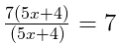(ii) 7p2q2(9r – 27) ÷ 63pq(r – 3)

=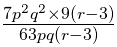= p2-1 q2-1 × 9 = 9pq

2. Divide as directed:
(i) 6(2x + 7) (5x – 3) ÷ 3(5x – 3)
(ii) 33pq (p + 3) (2q – 5) ÷ 11p (2q – 5)
Solution:

(i) 6(2x + 7) (5x – 3) ÷ 3(5x – 3)

=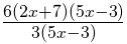= 2(2x + 7)

(ii) 33pq (p + 3) (2q – 5) ÷ 11p (2q – 5)

=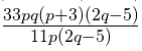= 3q(p + 3)

3. Factorise the expression and divide them as directed:
(i) (7x2 – 63x) ÷ 7(x – 3)
(ii) (3p2 + 17p + 10) ÷ (p + 5)
(iii) 10xy(14y2 + 43y – 21) ÷ 5x(7y – 3)
(iv) 12pqr(6p2 – 13pq + 6q2) ÷ 6pq(2p – 3q)
Solution:

(i) (7x2 – 63x) ÷ 7(x – 3)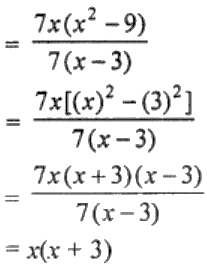(ii) (3p2 + 17p + 10) ÷ (p + 5)

= (3p2 + 17p + 10)/ (p + 5)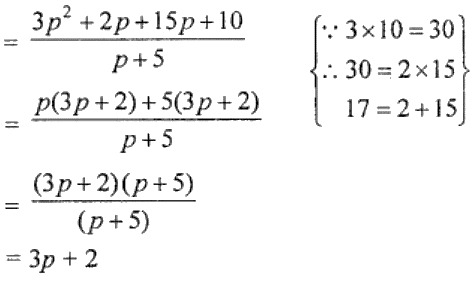(iii) 10xy(14y2 + 43y – 21) ÷ 5x(7y – 3)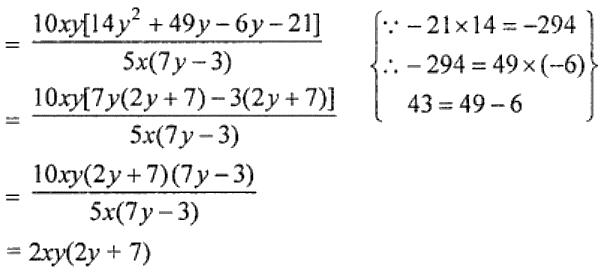(iv) 12pqr(6p2 – 13pq + 6q2) ÷ 6pq(2p – 3q)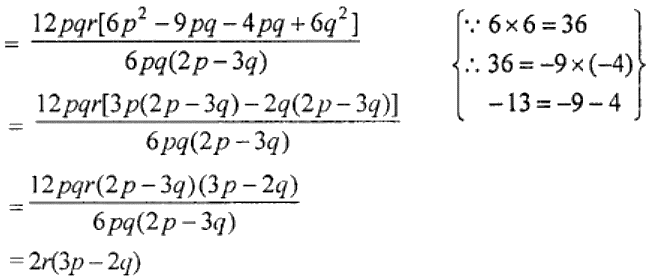1. Find the HCF of the given polynomials:
(i) 14pq, 28p2q2
(ii) 8abc, 24ab2, 12a2b
Solution:

(i) 14pq, 28p2q2

HCF of 14, 28 = 14

HCF of 14pq, 28p2q2 = 14pq

(ii) 8abc, 24ab2, 12a2b

HCF of 8, 24, 12 = 4

HCF of 8abc, 24ab2, 12a2b = 4ab

2. Factorise the following:
(i) 10x2 – 18x3 + 14x4
(ii) 5x2y + 10xyz + 15xy2
(iii) p2x2 + c2x2 – ac2 – ap2
(iv) 15(x + y)2 – 5x – 5y
(v) (ax + by)2 + (ay – bx)2
(vi) ax + by + cx + bx + cy + ay
(vii) 49x2 – 70xy + 25y2
(viii) 4a2 + 12ab + 9b2
(ix) 49p2 – 36q2
(x) 100x3 – 25xy2
(xi) x2 – 2xy + y2 – z2
(xii) x8 – y8
(xiii) 12x3 – 14x2 – 10x
(xiv) p2 – 10p + 21
(xv) 2x2 – x – 6
(xvi) 6x2 – 5xy – 6y2
(xvii) x2 + 2xy – 99y2
Solution:

(i) 10x2 – 18x3 + 14x4

HCF of 10, 18, 14 = 2

So, 10x2 – 18x3 + 14x4

= 2x2 (5 – 9x + 7x2)

(ii) 5x2y + 10xyz + 15xy2

HCF of 5, 10, 15 = 5

So, 5x2y + 10xyz + 15xy2

= 5xy (x + 2z + 3y)

(iii) p2x2 + c2x2 – ac2 – ap

= p2x2 – ap2 + c2x2 – ac2

= p2(x2 – a) + c2(x2 – a)

= (x2 – a) (p2 + c2)

(iv) 15(x + y)2 – 5x – 5y

= 15(x + y)2 – 5(x + y)

= 5(x + y) [3(x + y) – 1]

= 5(x + y) (3x + 3y – 1)

(v) (ax + by)2 + (ay – bx)2

On expanding, we have

= a2x2 + b2y2 + 2abxy + a2y2 + b2x2 – 2abxy

= a2x2 + a2y2 + b2x2 + b2y2

= a2(x2 + y2) + b2(x2 + y2)

= (x2 + y2) (a2 + b2)

(vi) ax + by + cx + bx + cy + ay

= ax + bx + cx + ay + by + cy [On grouping the like variables]

= x(a + b + c) + y(a + b + c)

= (a + b + c) (x + y)

(vii) 49x2 – 70xy + 25y2

= (7x)2 – 2 × 7x × 5y + (5y)2 [∵ (a – b)2 = a2 – 2ab + b2]

= (7x – 5y)2

(viii) 4a2 + 12ab + 9b2

= (2a)22 + 2 × 2a × 3b + (3b)2 [∵ (a + b)2 = a2 + 2ab + b2]

= (2a + 3b)2

(ix) 49p2 – 36q2

= (7p)2 – (6q)2

= (7p + 6q) (7p – 6q) [∵ a2 – b2 = (a + b) (a – b)]

(x) 100x3 – 25xy2

= 25x(x2 – y2) = 25x{(x)2 – (y)2}

= 25x(x + y) (x – y)

(xi) x2 – 2xy + y2 – z2

= (x – y)2 – (z)2 [∵ a2 -2ab + b2 = (a – b)2 and a2 – b2 = (a + b) (a – b)]

= (x – y + z)(x – y – z)

(xii) x8 – y8

= (x4)2 – (y4)2 [∵ a2 – b2 = (a + b)(a- b)]

= (x4 + y4) (x4 – y4)

= (x4 + y4) [(x2)2 – (y2)2]

= (x4 + y4) (x2 + y2) (x2 – y2)

= (x4 + y4 (x2 + y2) (x + y) (x – y)

(xiii) 12x3 – 14x2 – 10x

= 2x(6x2 – 7x – 5) [Now, as 6 × (-5) = -30 ⇒ -30 = -10 × 3 and -7 = -10 + 3]

= 2x(6x2 + 3x – 10x – 5)

= 2x{3x(2x + 1) – 5(2x + 1)}

= 2x(2x + 1) (3x – 5)

(xiv) p2 – 10p + 21

= p2 – 3p – 7p + 21 [Now, as 21 =-3 × (-7) and -10 = -3 – 7]

= p(p – 3) – 7(p – 3)

= (p – 3)(p – 7)

(xv) 2x2 – x – 6

= 2x2 – 4x + 3x – 6 [Now, as -6 × 2 = -12 ⇒ -12 = -4 × 3 and -1 = -4 + 3]

= 2x (x – 2) + 3(x – 2)

= (x – 2) (2x + 3)

(xvi) 6x2 – 5xy – 6y2

= 6x2 – 9xy + 4xy – 6y2 [Now, as 6 × (-6) = -36 ⇒ – 36 = -9 × 4 and – 5 = -9 + 4]

= 3x(2x – 3y) + 2y(2x – 3y)

= (2x – 3y) (3x + 2y)

(xvii) x2 + 2xy – 99y2

= x2 + 11xy – 9xy – 99y2 [Now, as -99 = -11 × 9 and -2 = -11 + 9 }

= x(x + 11y) – 9y(x + 11y)

= (x + 11y) (x – 9y)

3. Divide as directed:
(i) 15(y + 3)(y2 – 16) ÷ 5(y2 – y – 12)
(ii) (3x3 – 6x2 – 24x) ÷ (x – 4) (x + 2)
(iii) (x4 – 81) ÷ (x3 + 3x2 + 9x + 27)
Solution:

(i) 15(y + 3)(y2 – 16) ÷ 5(y2 – y – 12)

y2 – 16 = (y)2 – (4)2

= (y + 4)(y – 4)

y2 – y – 12 = y2 – 4y + 3y – 12

= y(y – 4) + 3(y – 4)

= (y – 4)(y + 3)

Now,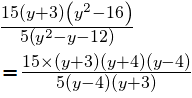= 3(y + 4)

(ii) (3x3 – 6x2 – 24x) ÷ (x – 4) (x + 2)

3x3 – 6x2 – 24x = 3x(x2 – 2x – 8)

= 3x{x2 – 4x + 2x – 8}

= 3x{x(x – 4) + 2(x – 4)}

= 3x(x – 4) (x + 2)

Now,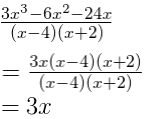(iii) (x4 – 81) ÷ (x3 + 3x2 + 9x + 27)

x4 – 81 = (x2)2 – (9)2 = (x2 + 9) (x2 – 9)

= (x2 + 9) [(x)2 – (3)2]

= (x2 + 9) (x + 3) (x – 3)

And,

x3 + 3x2 + 9x + 27 = (x)2 + (x + 3) + 9 (x + 3)

= (x2 + 9) (x + 3)

Now,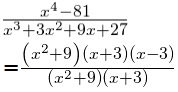= (x – 3)